# RD Sharma Solutions for Class 11 Chapter 18 - Binomial Theorem Exercise 18.2

This exercise discusses problems based on the general term and middle terms in a binomial expansion. The solutions provided here are explained in detail, which helps students to gain confidence and make their exam preparation more effective. Experts at BYJU’S have provided solutions to an ample number of questions, which are present in RD Sharma textbook. These solutions boost confidence among students to perform very well in their upcoming board exams. Students can access and download RD Sharma Class 11 Maths Solutions for free from the links given below.

## Download the pdf of RD Sharma Solutions for Class 11 Maths Exercise 18.2 Chapter 18 – Binomial Theorem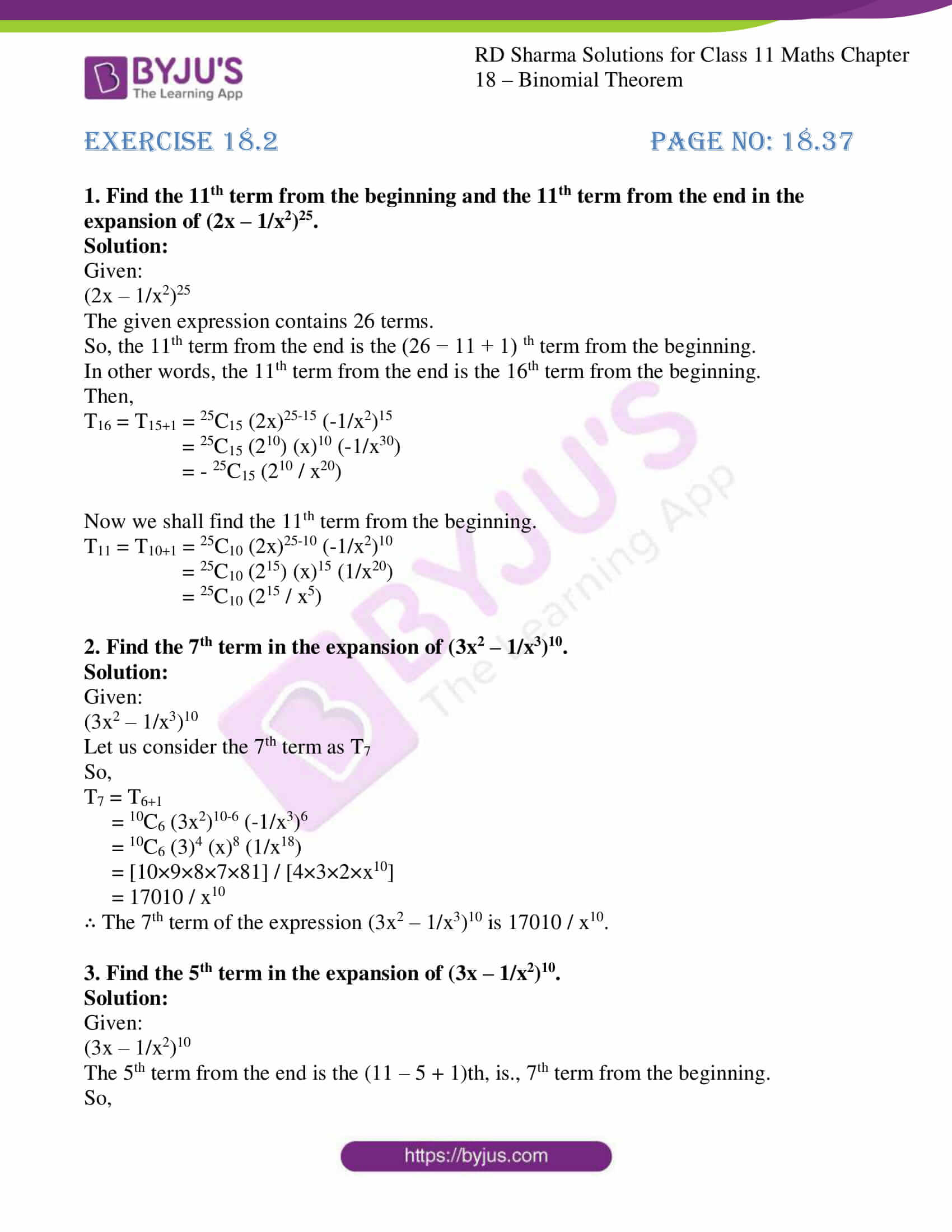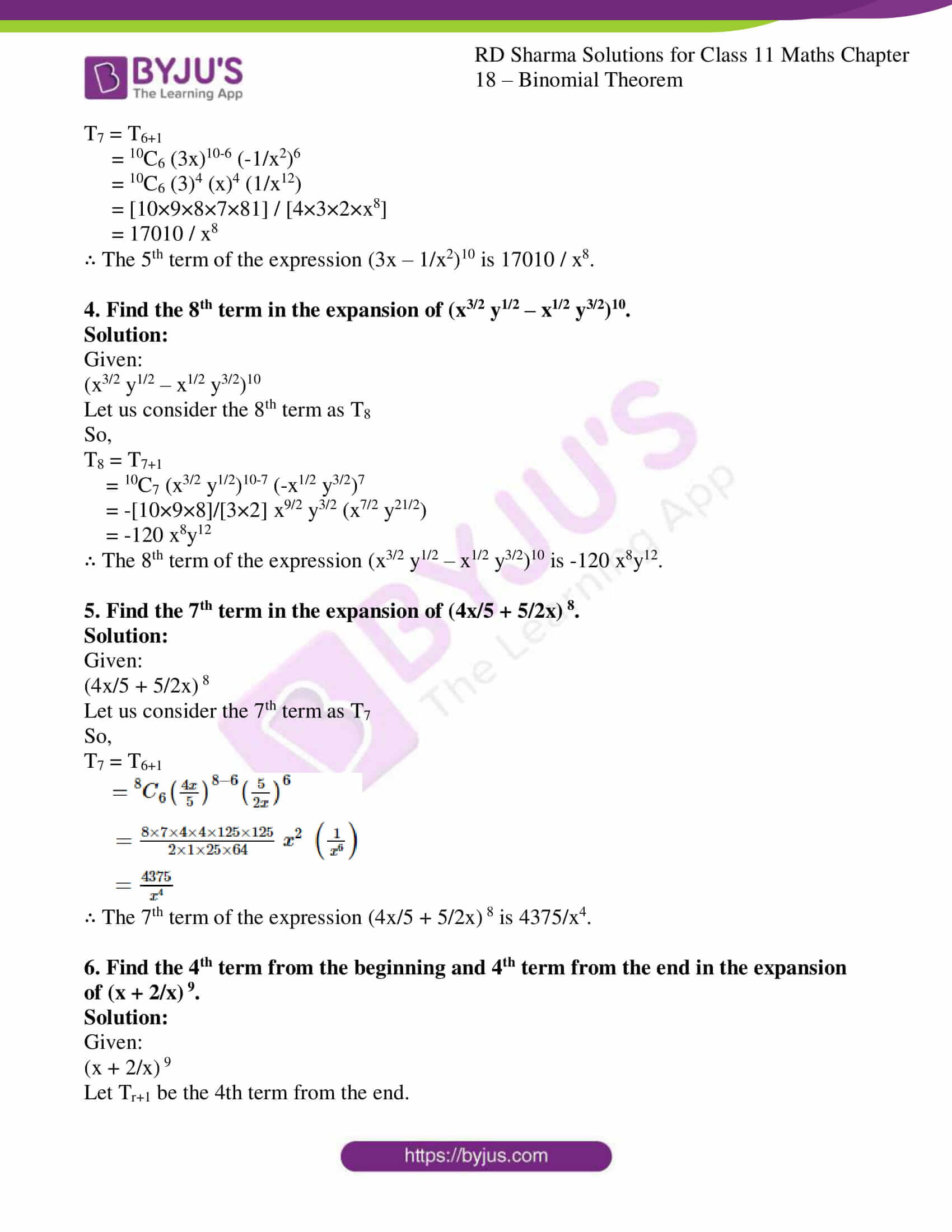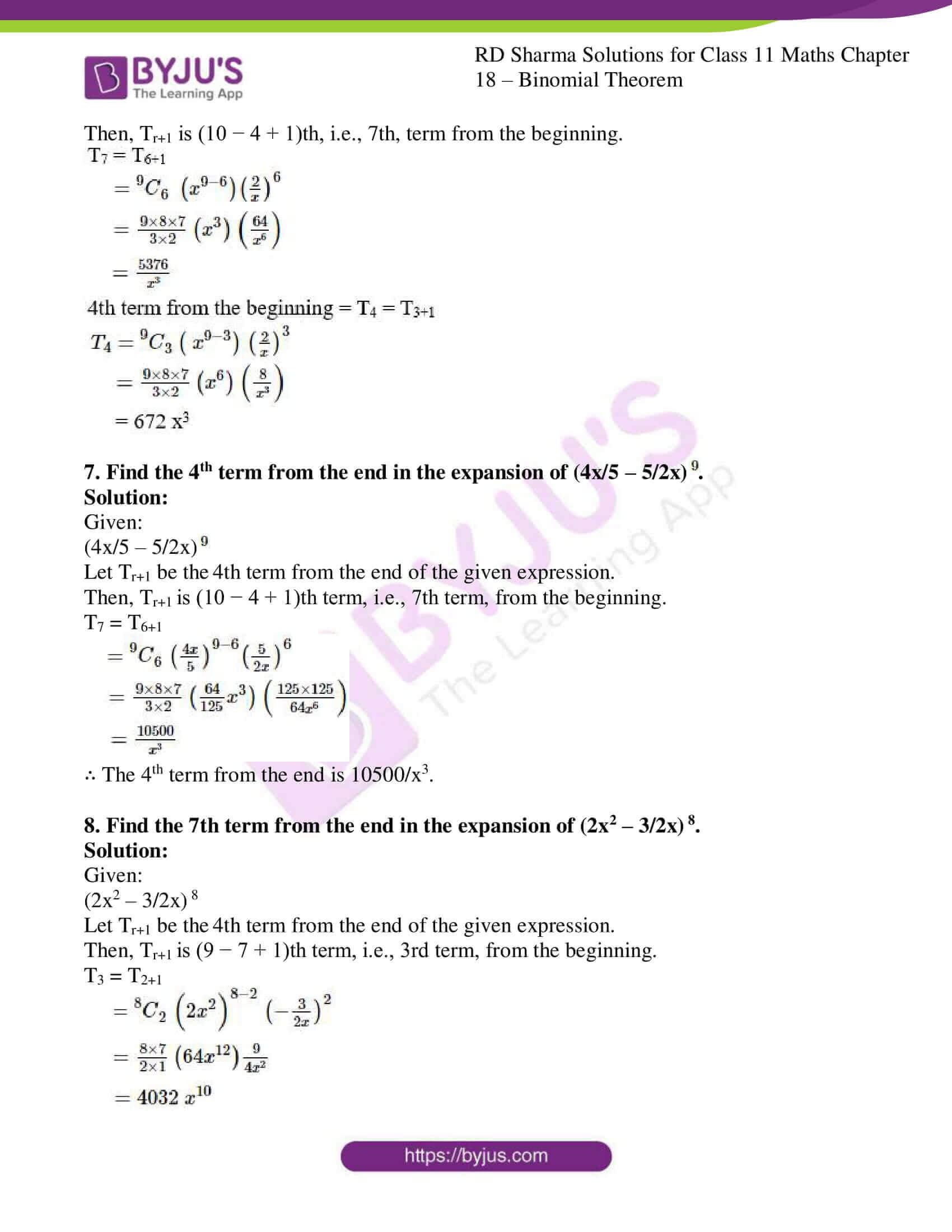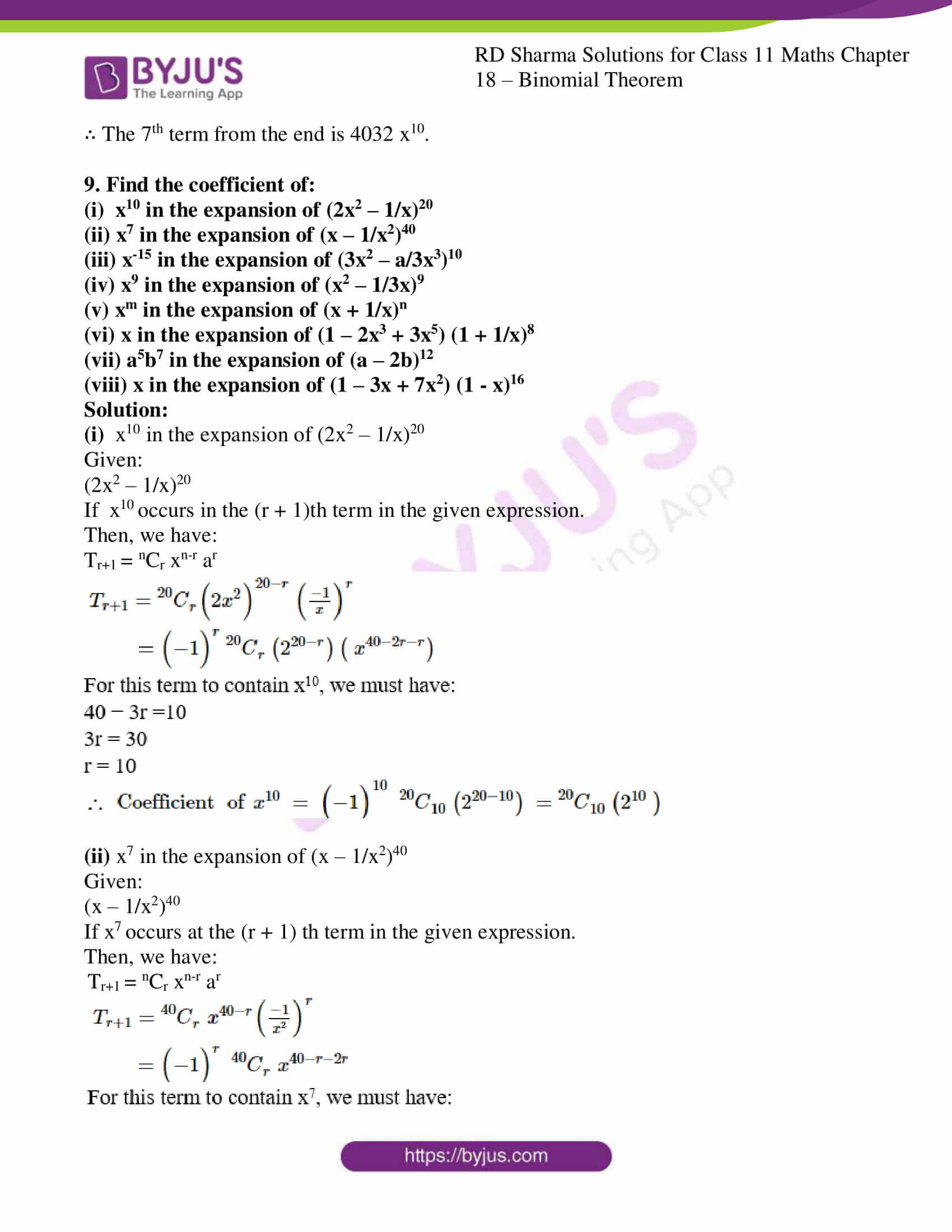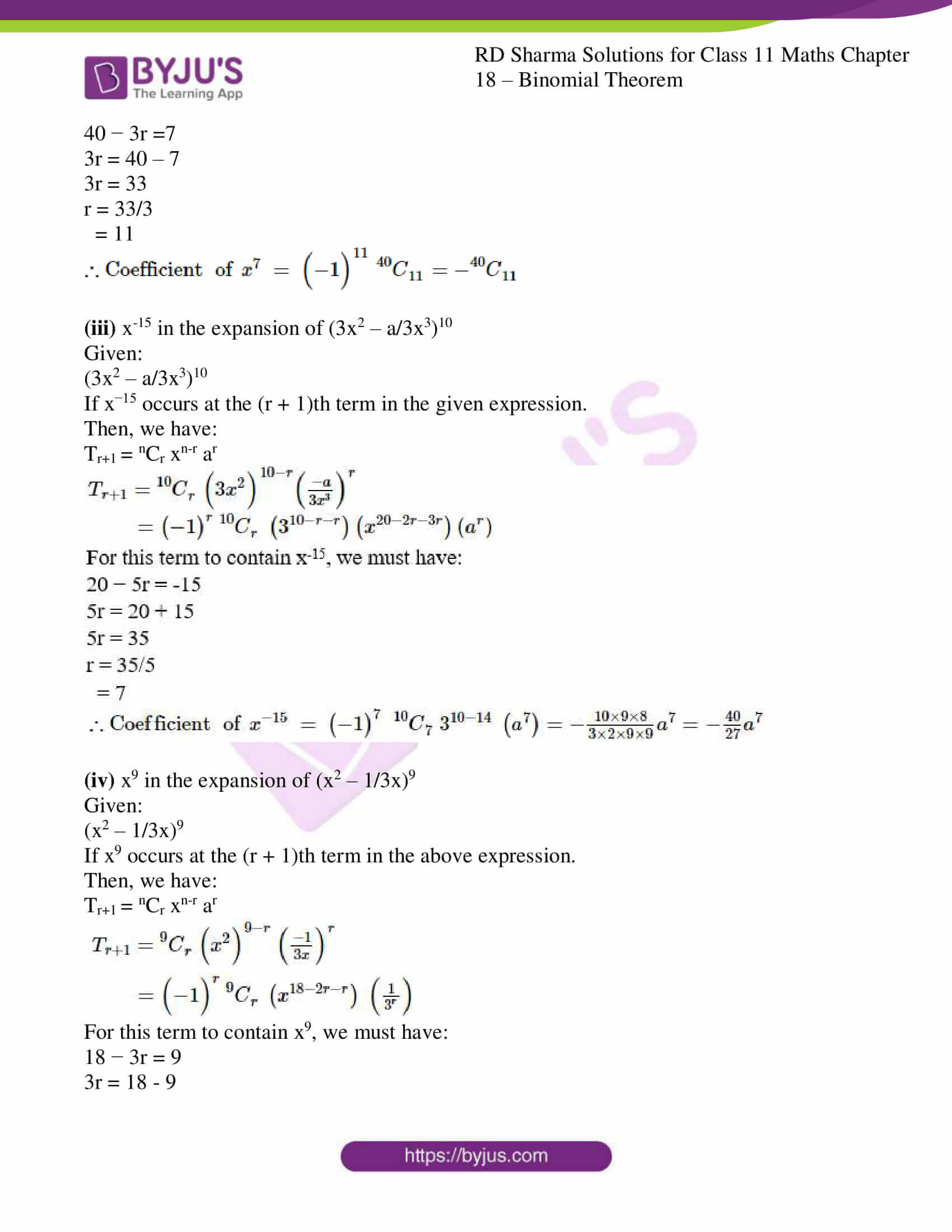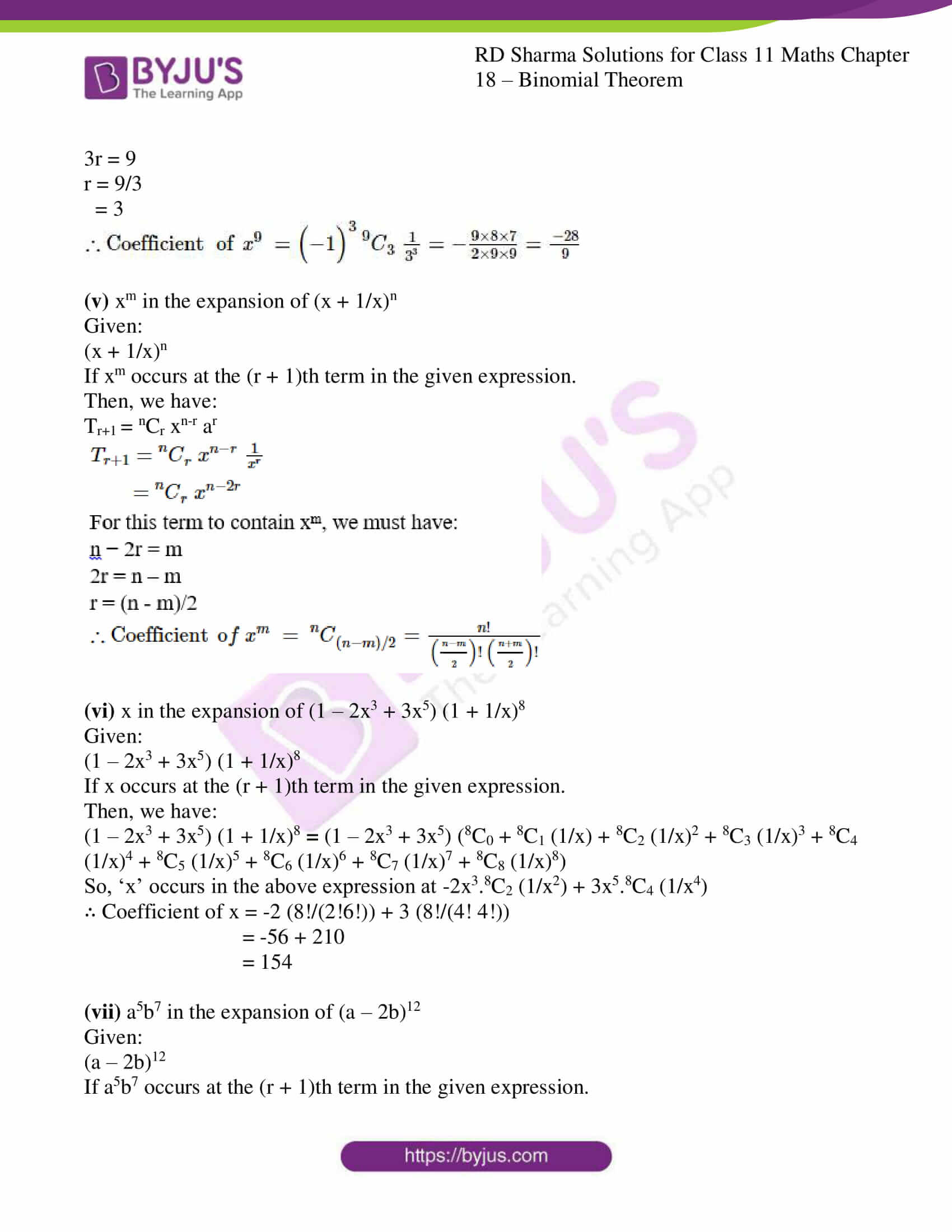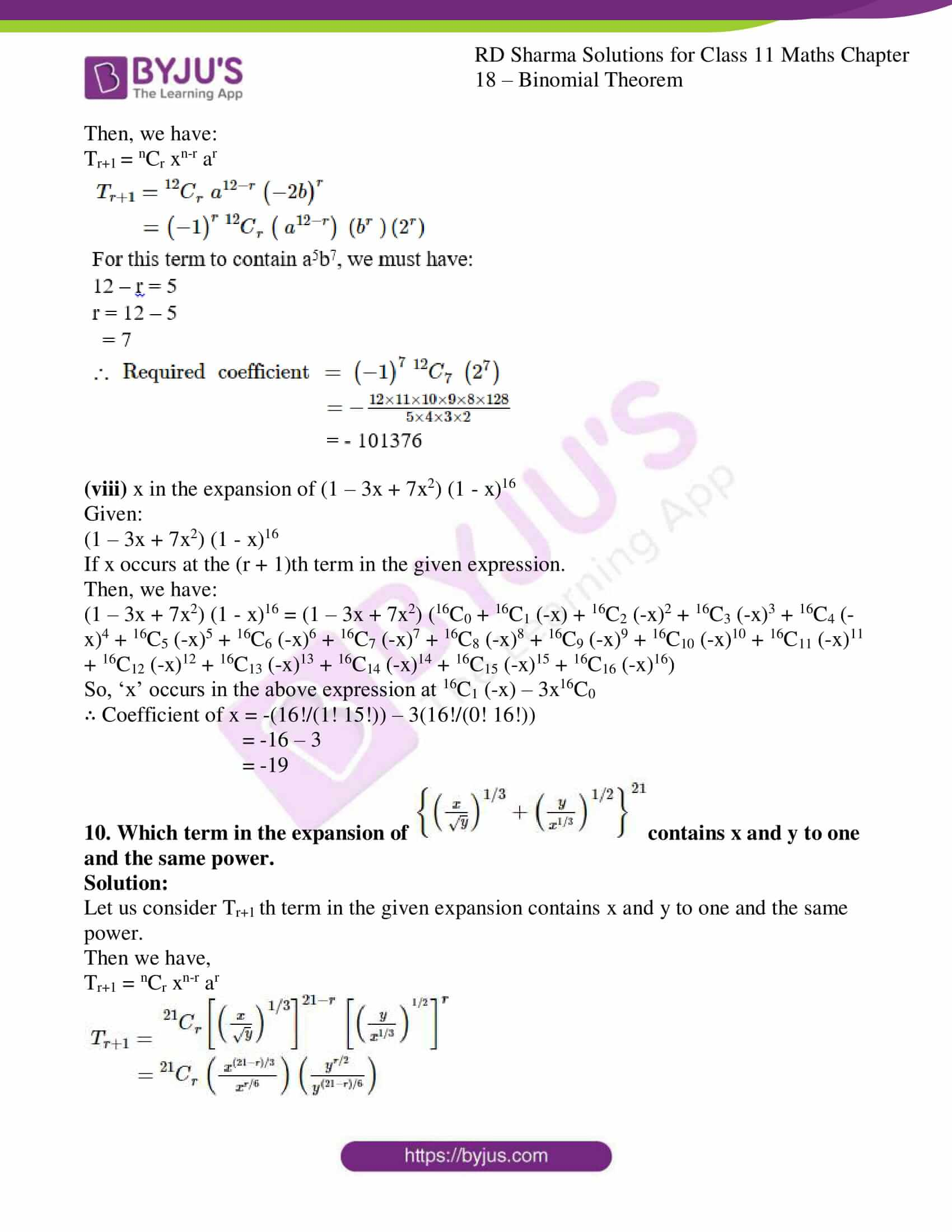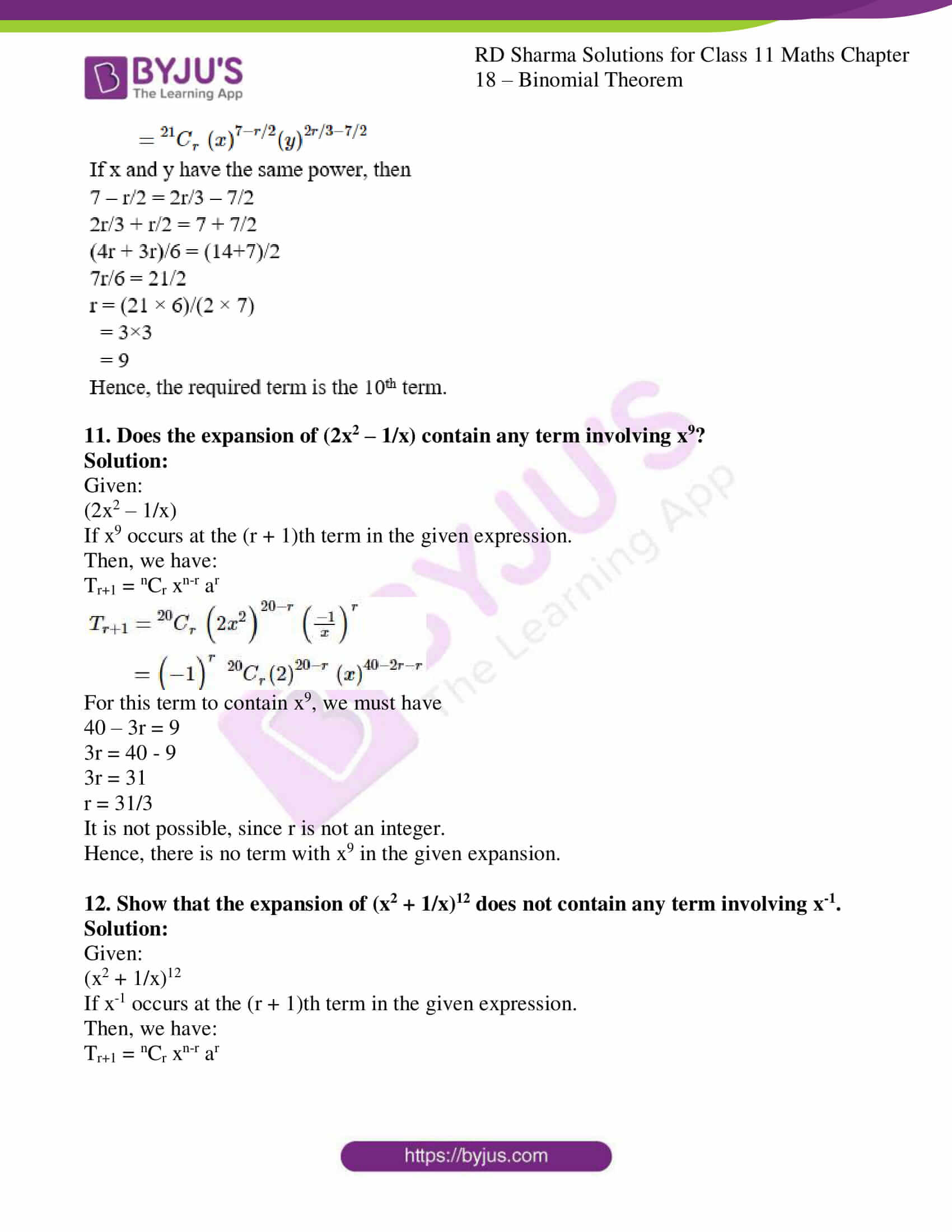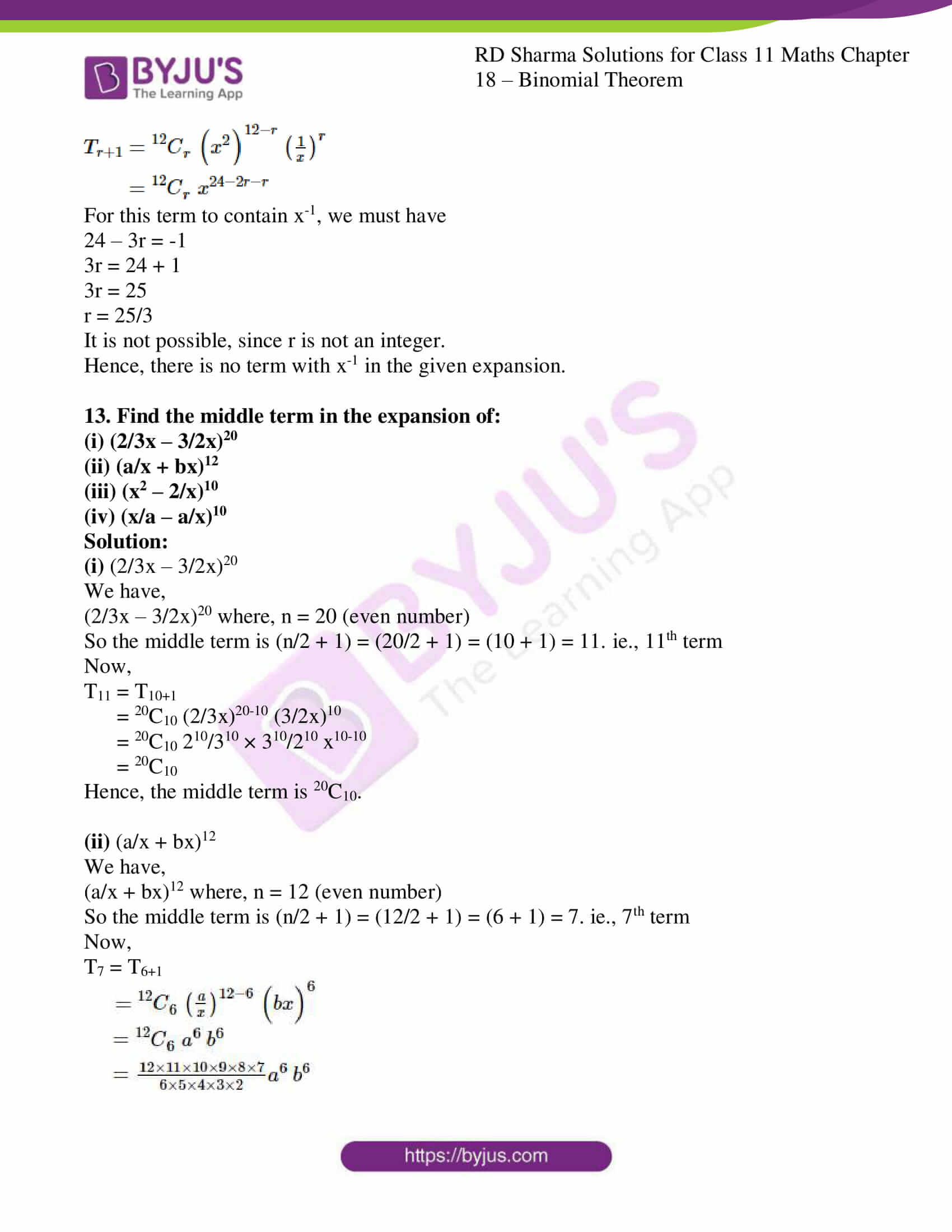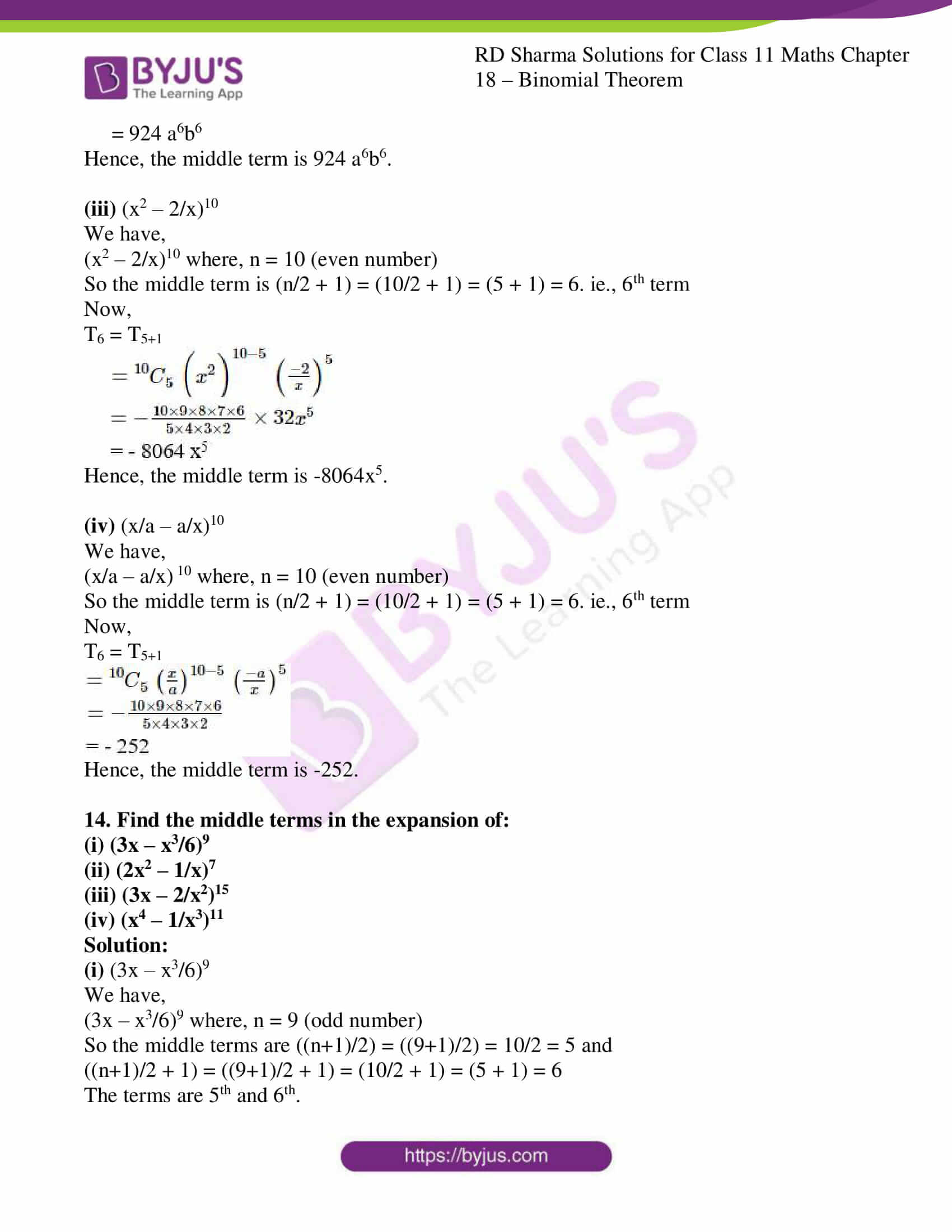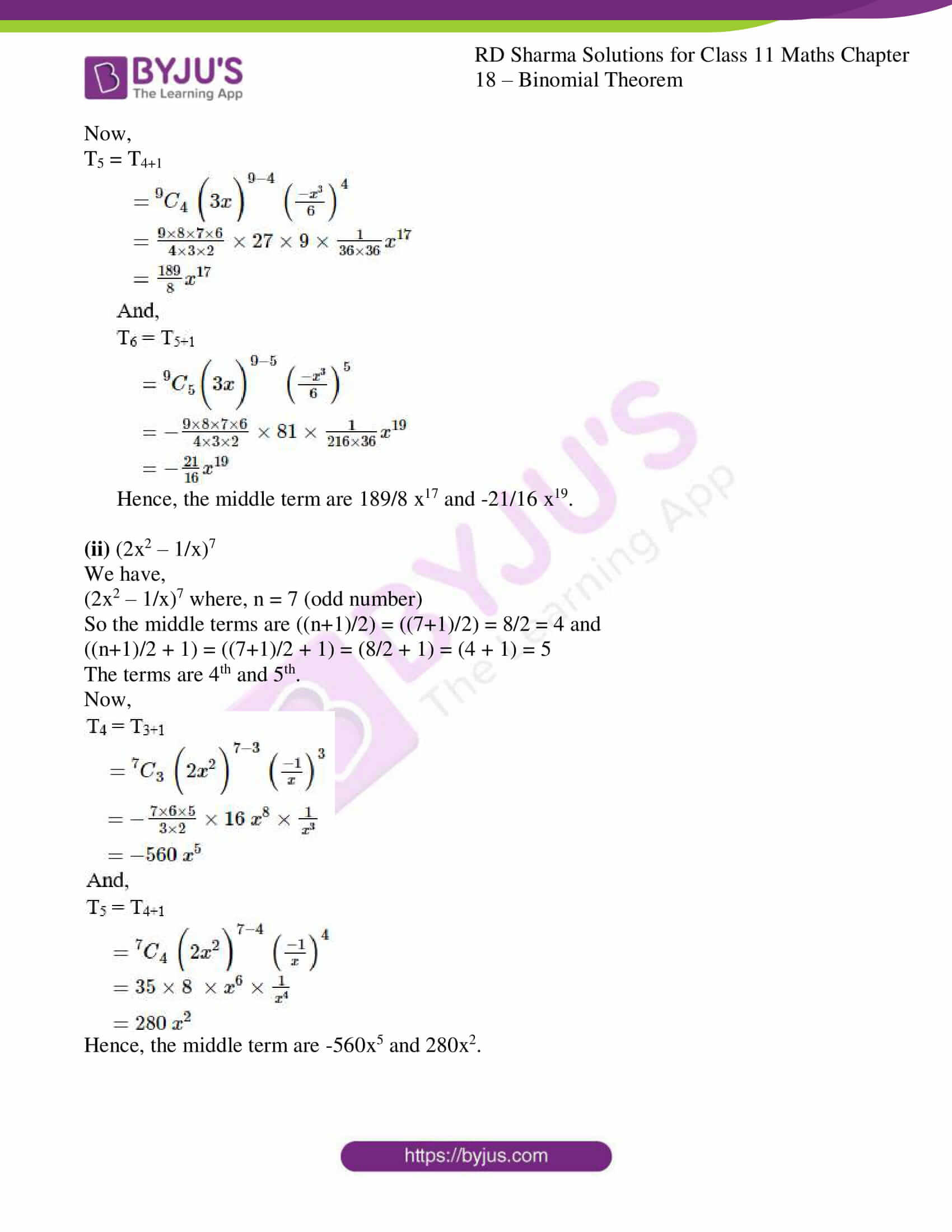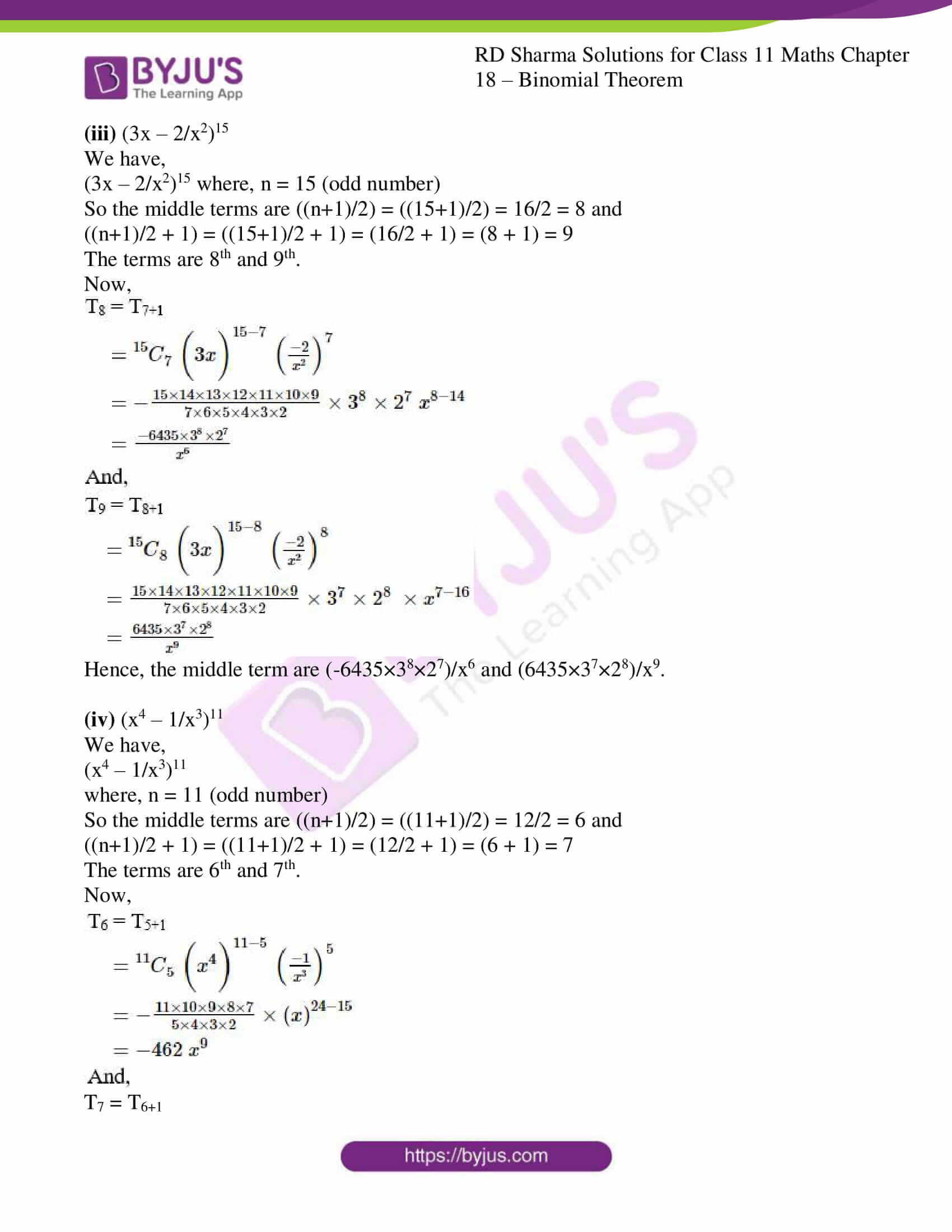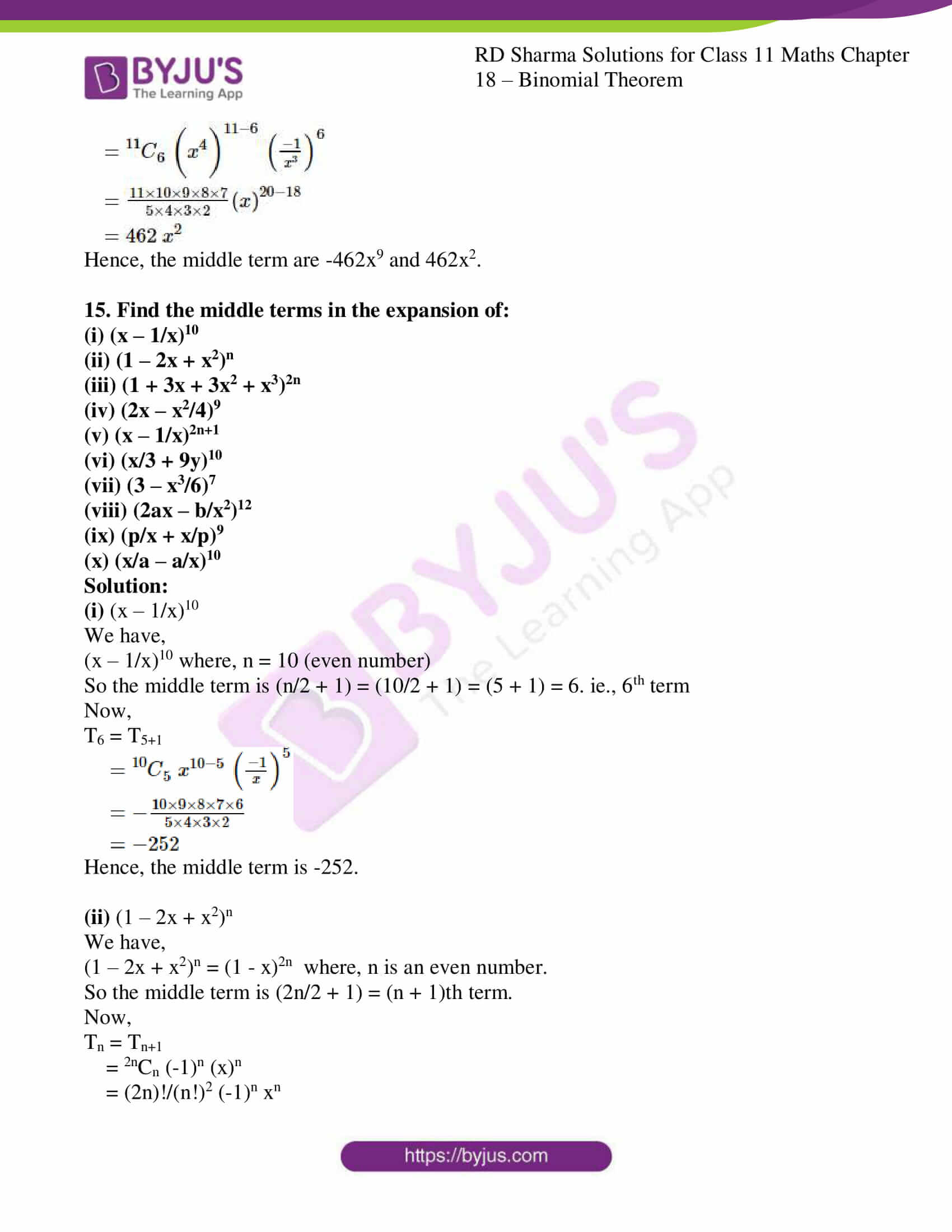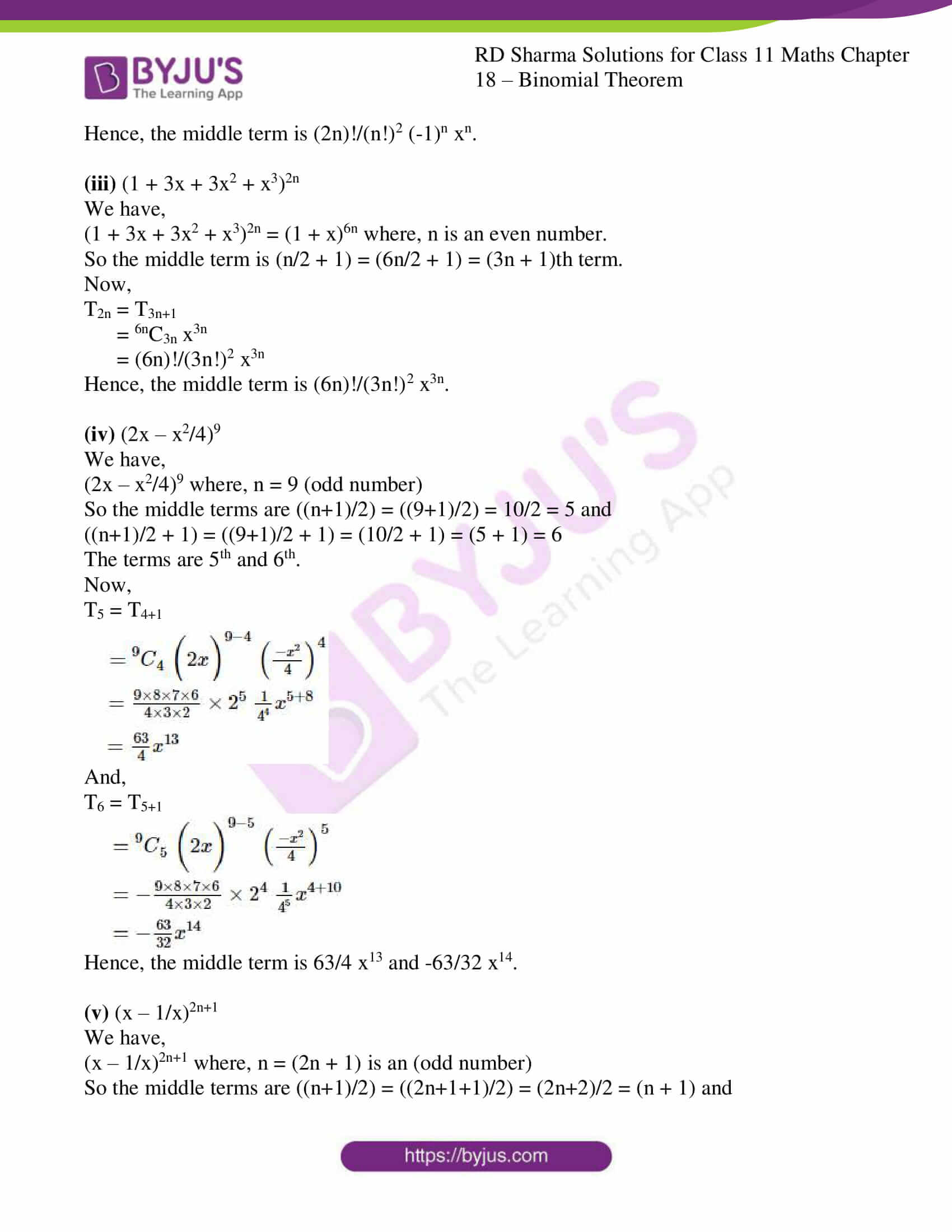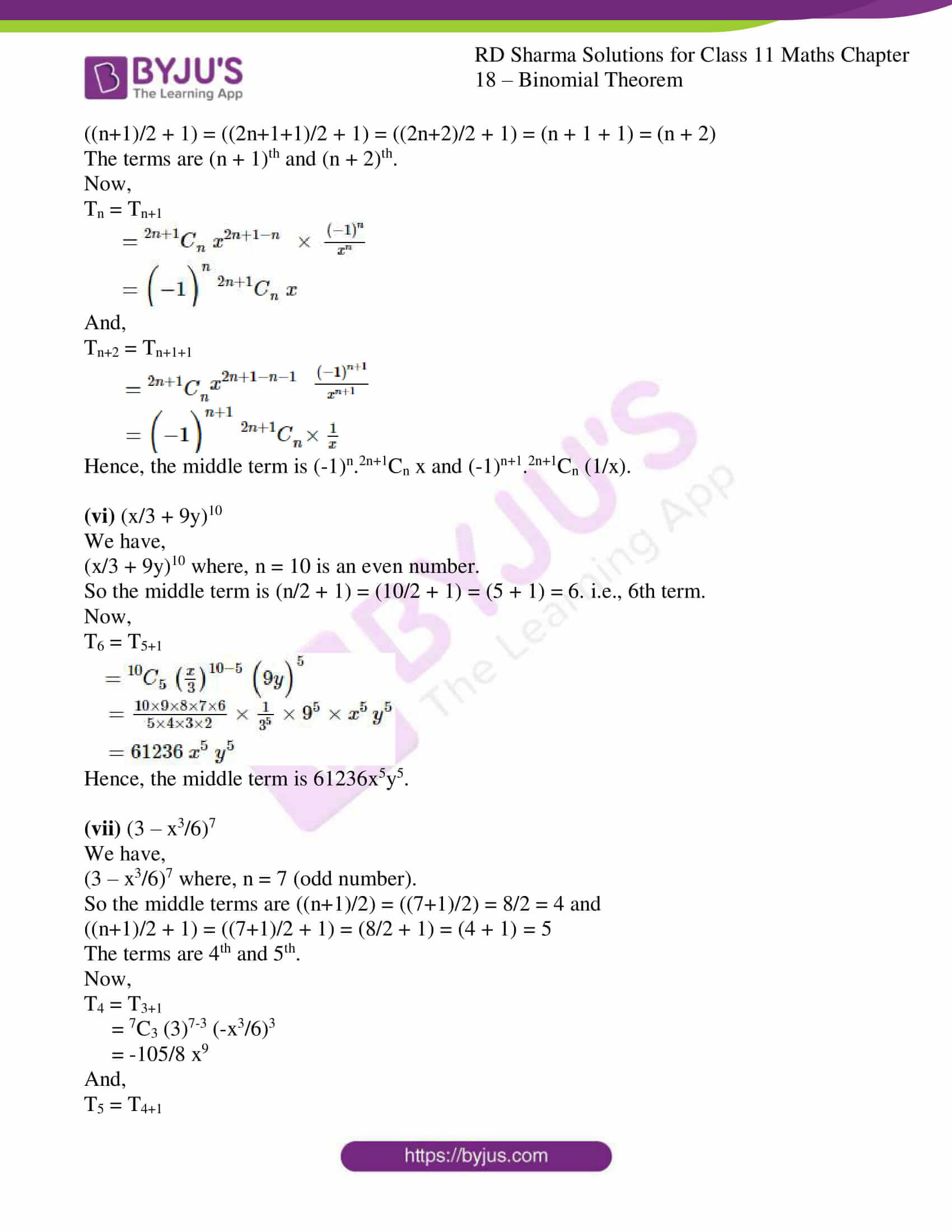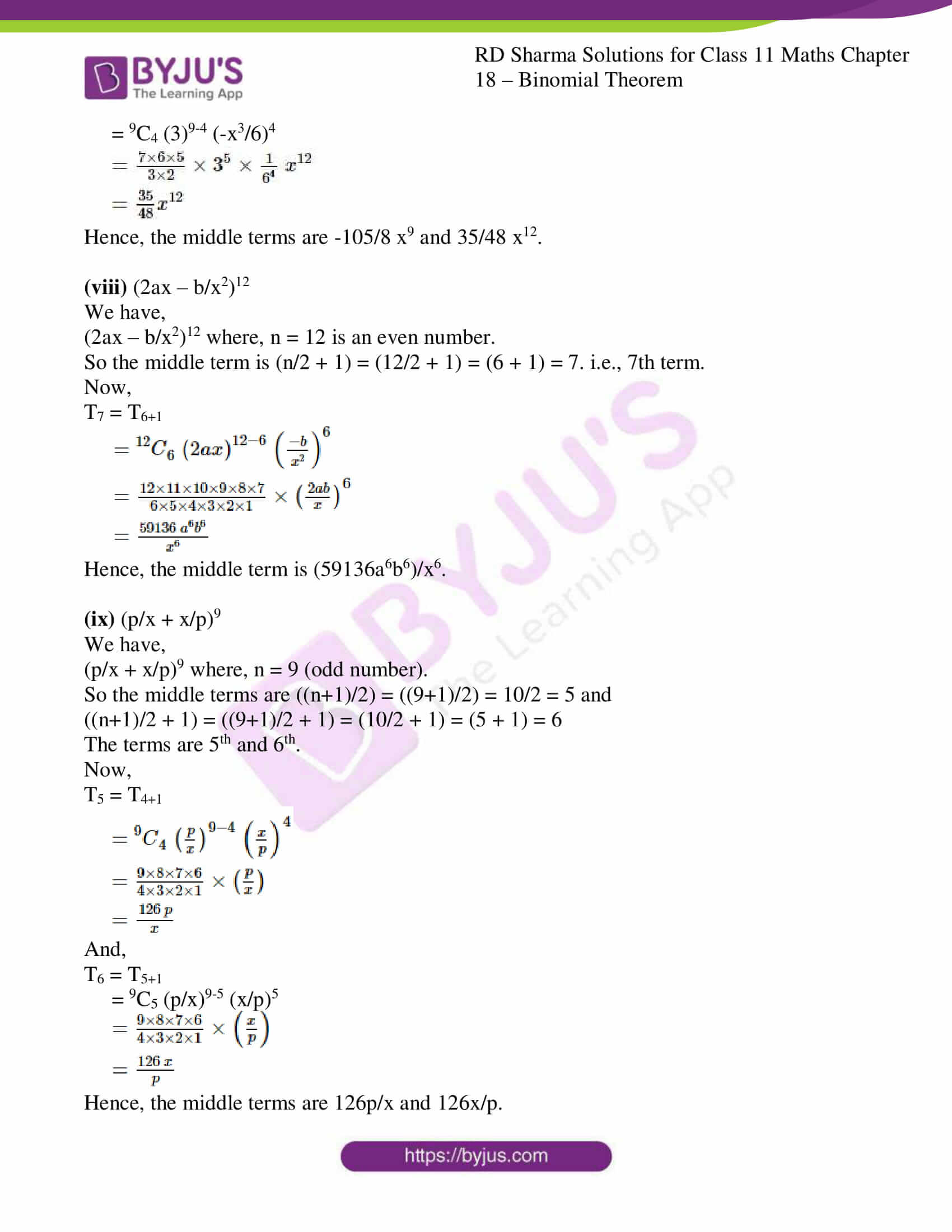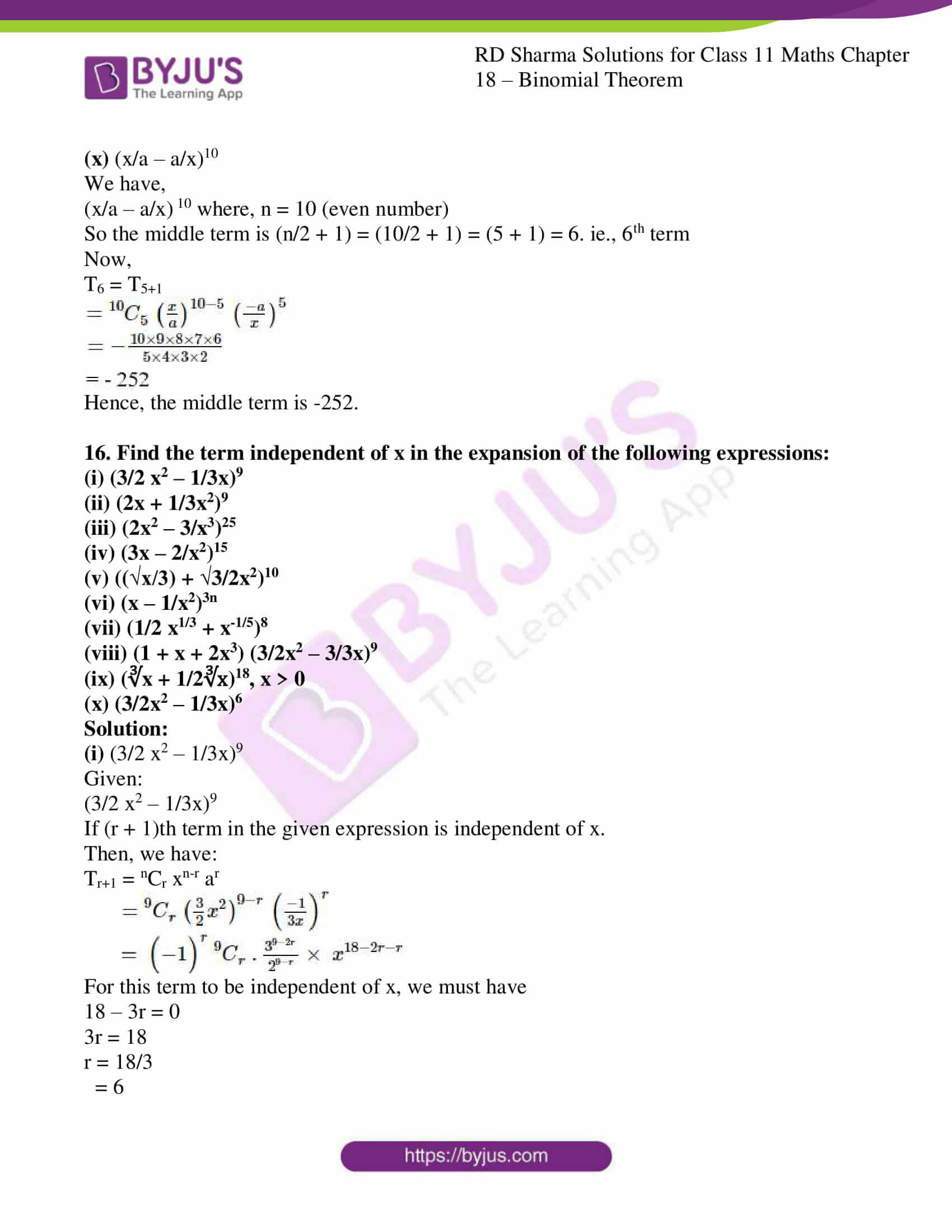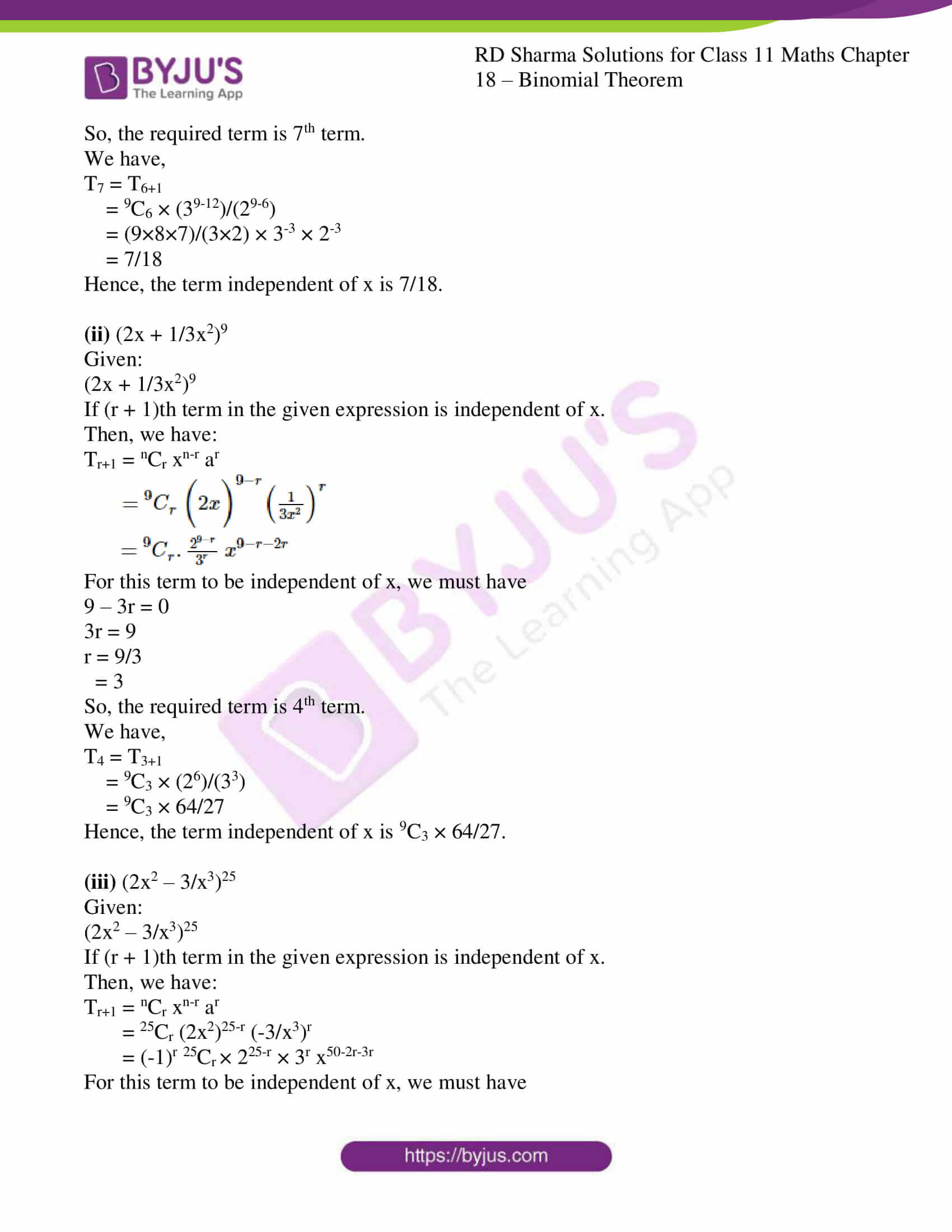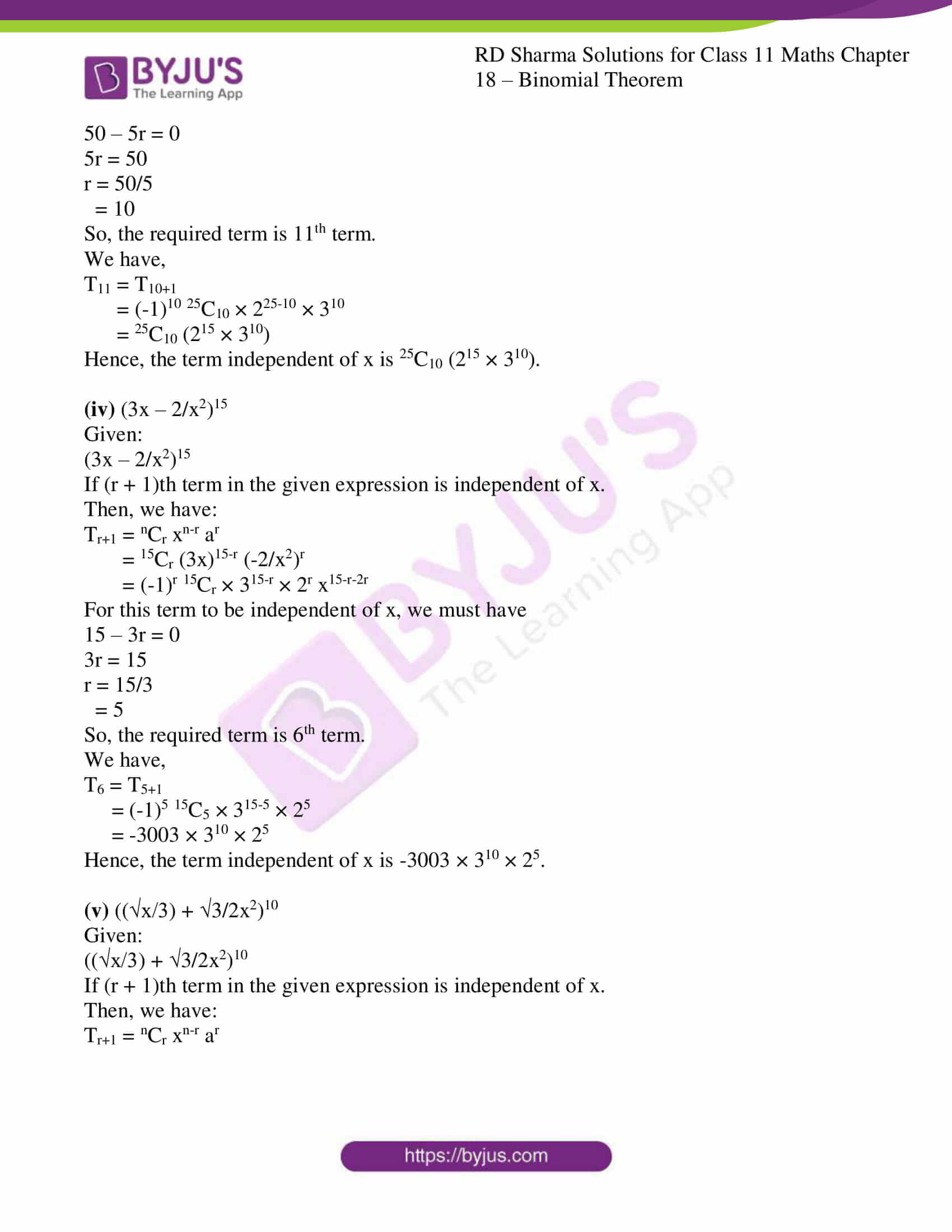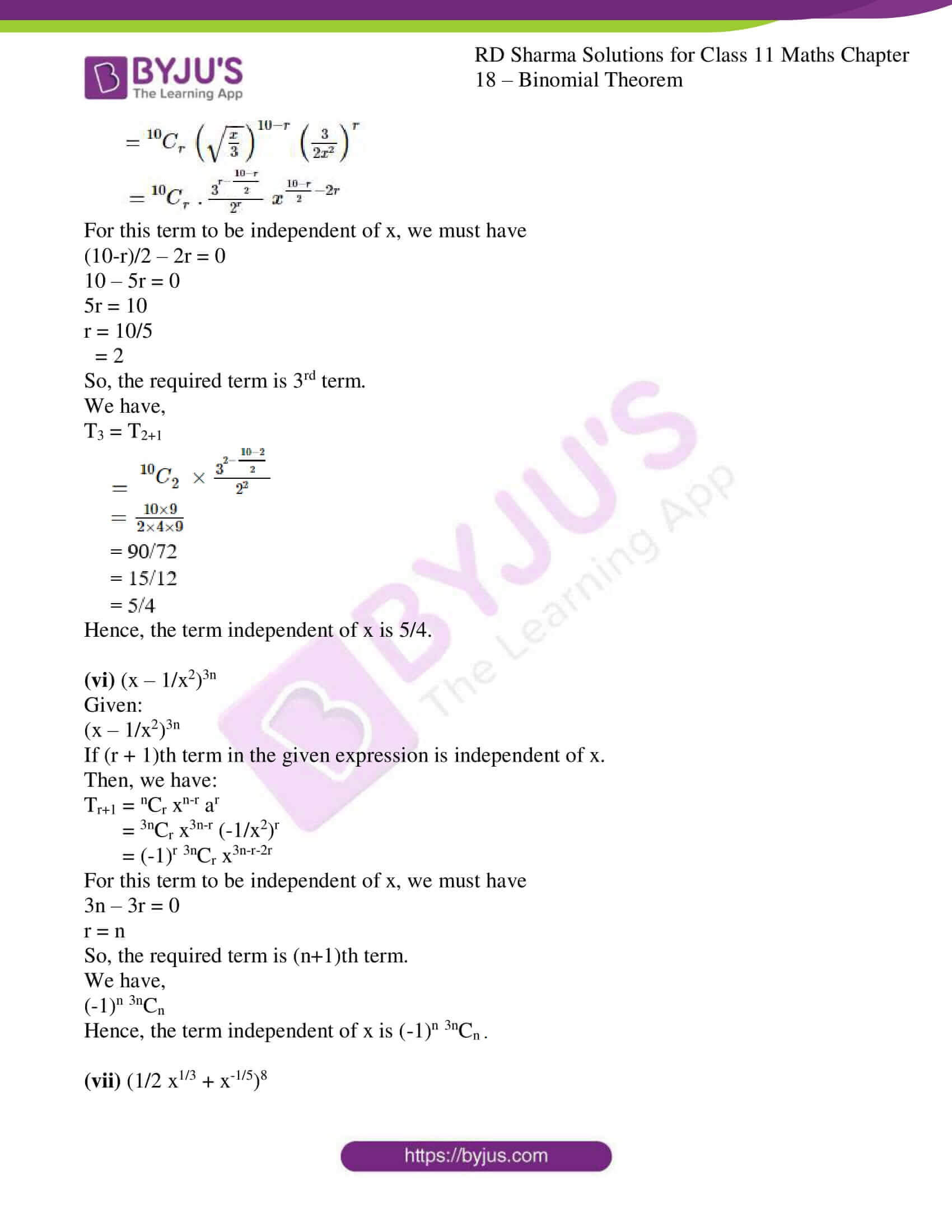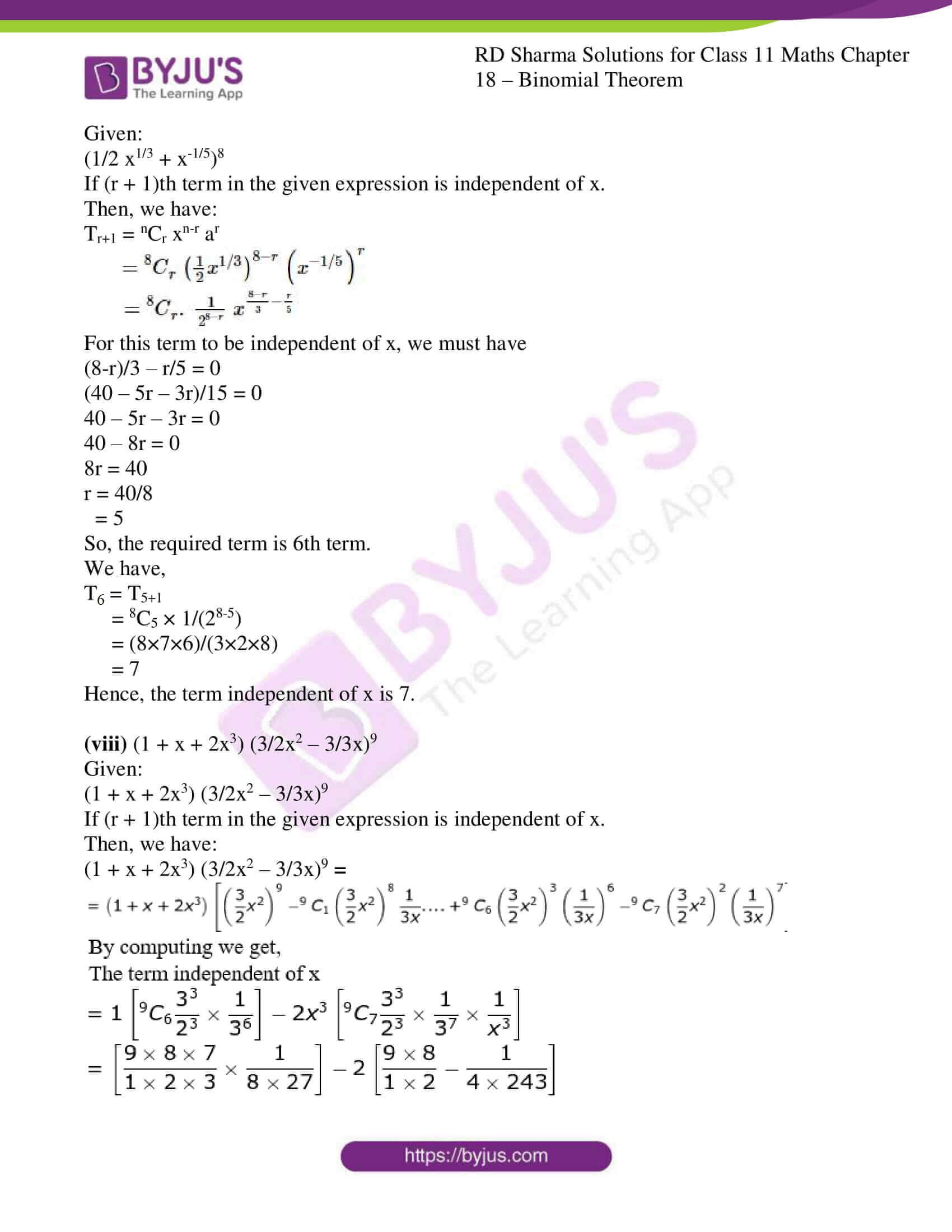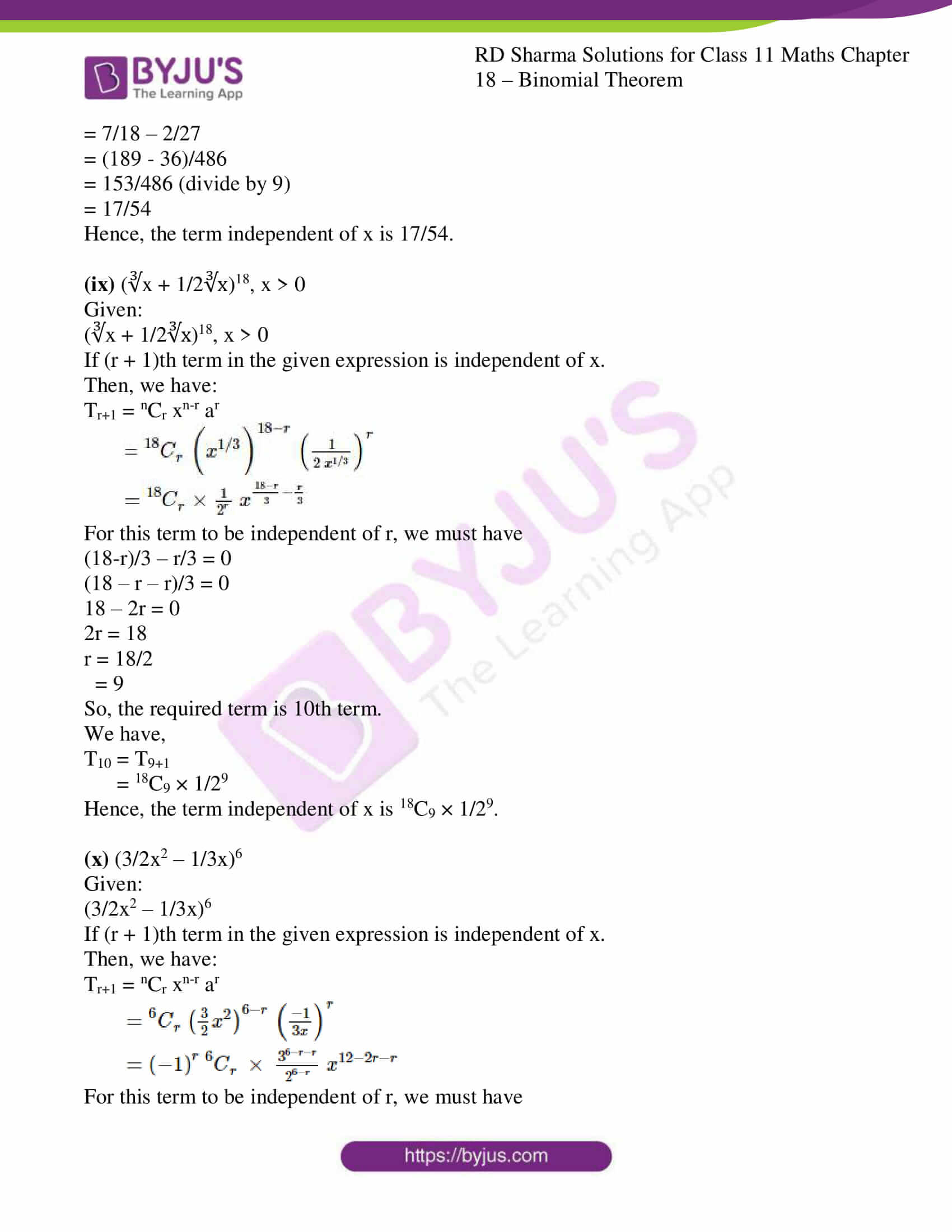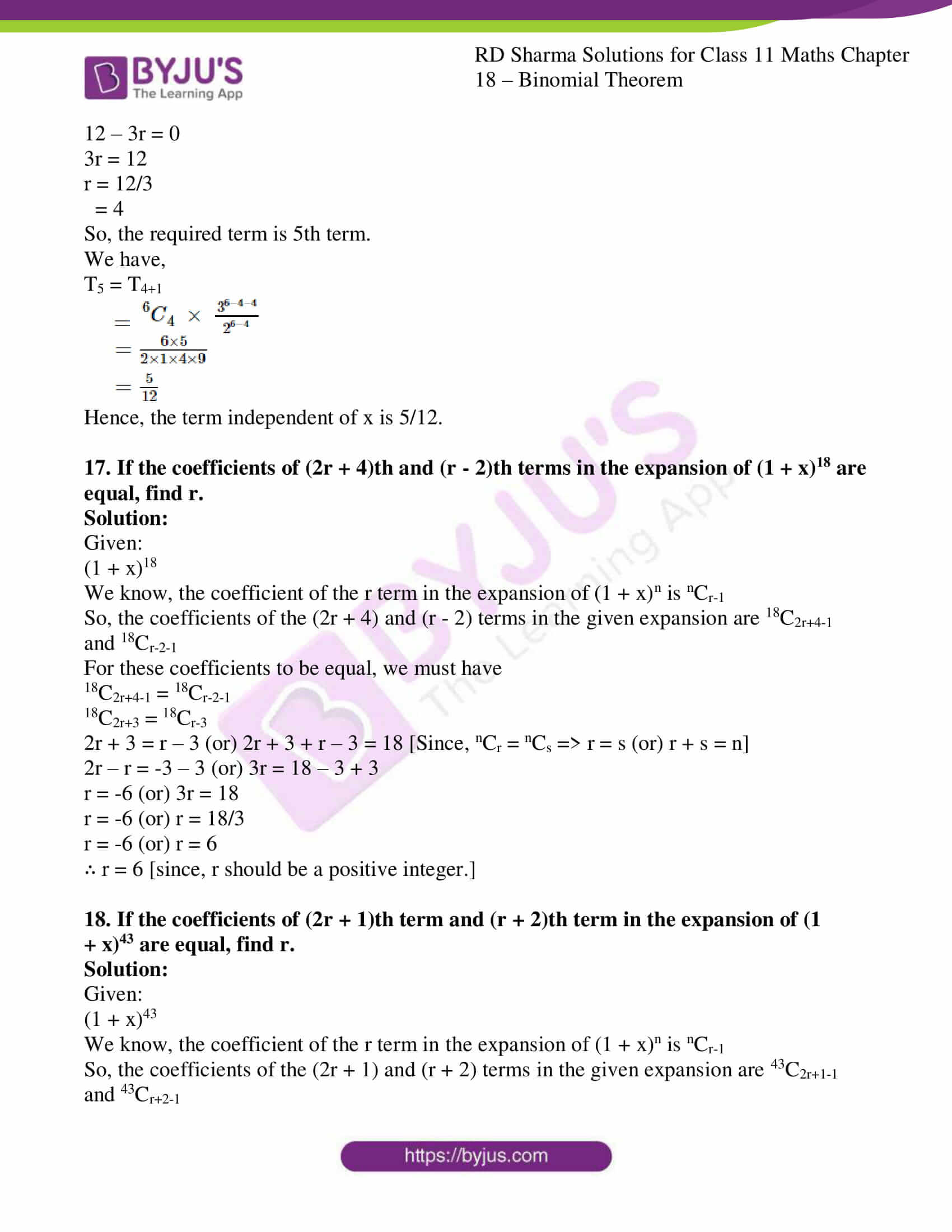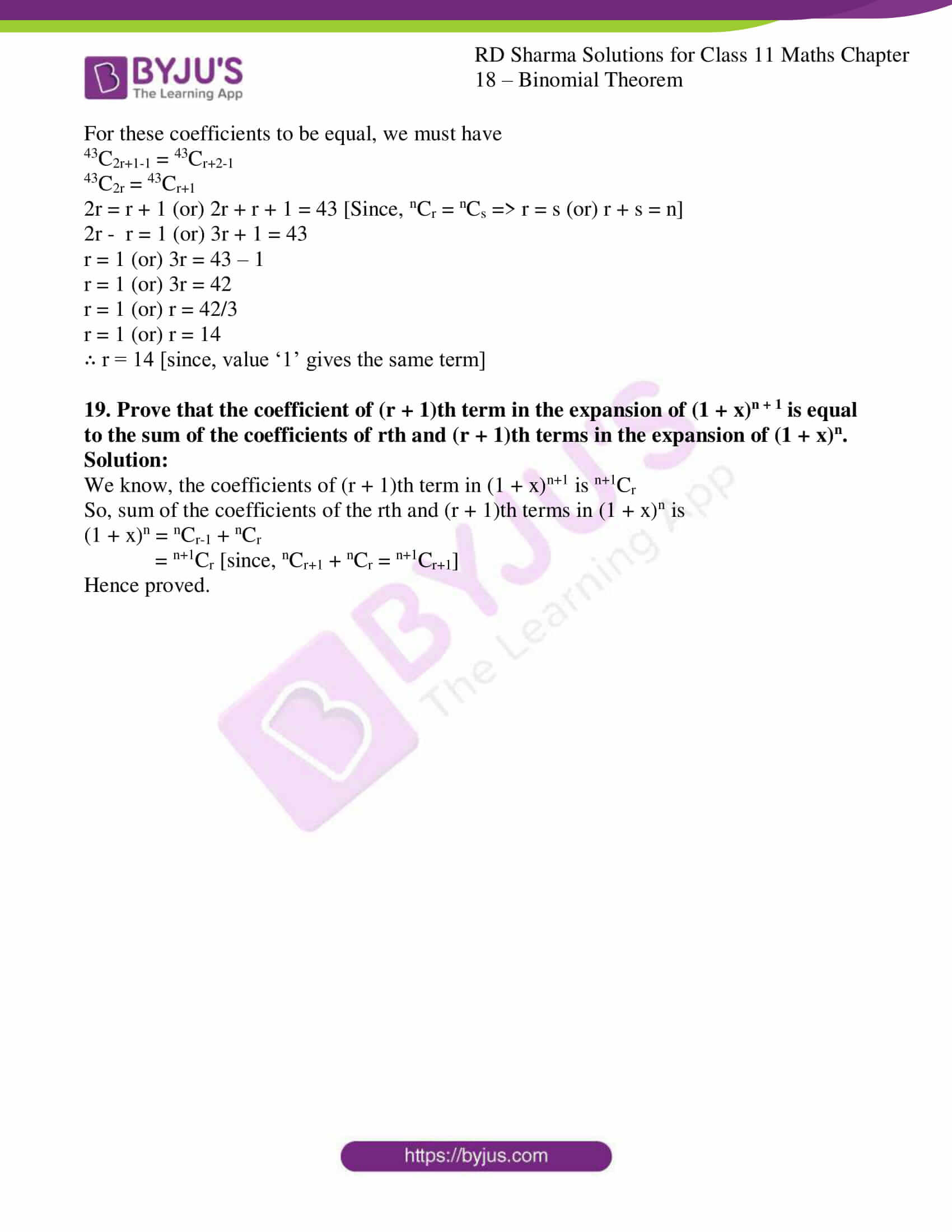### Also, access another exercise of RD Sharma Solutions for Class 11 Maths Chapter 18 – Binomial Theorem

Exercise 18.1 Solutions

### Access answers to RD Sharma Solutions for Class 11 Maths Exercise 18.2 Chapter 18 – Binomial Theorem

1. Find the 11th term from the beginning and the 11th term from the end in the expansion of (2x – 1/x2)25.

Solution:

Given:

(2x – 1/x2)25

The given expression contains 26 terms.

So, the 11th term from the end is the (26 − 11 + 1) th term from the beginning.

In other words, the 11th term from the end is the 16th term from the beginning.

Then,

T16 = T15+1 = 25C15 (2x)25-15 (-1/x2)15

= 25C15 (210) (x)10 (-1/x30)

= – 25C15 (210 / x20)

Now we shall find the 11th term from the beginning.

T11 = T10+1 = 25C10 (2x)25-10 (-1/x2)10

= 25C10 (215) (x)15 (1/x20)

= 25C10 (215 / x5)

2. Find the 7th term in the expansion of (3x2 – 1/x3)10.

Solution:

Given:

(3x2 – 1/x3)10

Let us consider the 7th term as T7

So,

T7 = T6+1

= 10C6 (3x2)10-6 (-1/x3)6

= 10C6 (3)4 (x)8 (1/x18)

= [10×9×8×7×81] / [4×3×2×x10]

= 17010 / x10

∴ The 7th term of the expression (3x2 – 1/x3)10 is 17010 / x10.

3. Find the 5th term in the expansion of (3x – 1/x2)10.

Solution:

Given:

(3x – 1/x2)10

The 5th term from the end is the (11 – 5 + 1)th, is., 7th term from the beginning.

So,

T7 = T6+1

= 10C6 (3x)10-6 (-1/x2)6

= 10C6 (3)4 (x)4 (1/x12)

= [10×9×8×7×81] / [4×3×2×x8]

= 17010 / x8

∴ The 5th term of the expression (3x – 1/x2)10 is 17010 / x8.

4. Find the 8th term in the expansion of (x3/2 y1/2 – x1/2 y3/2)10.

Solution:

Given:

(x3/2 y1/2 – x1/2 y3/2)10

Let us consider the 8th term as T8

So,

T8 = T7+1

= 10C7 (x3/2 y1/2)10-7 (-x1/2 y3/2)7

= -[10×9×8]/[3×2] x9/2 y3/2 (x7/2 y21/2)

= -120 x8y12

∴ The 8th term of the expression (x3/2 y1/2 – x1/2 y3/2)10 is -120 x8y12.

5. Find the 7th term in the expansion of (4x/5 + 5/2x) 8.

Solution:

Given:

(4x/5 + 5/2x) 8

Let us consider the 7th term as T7

So,

T7 = T6+1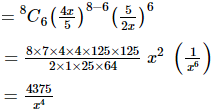∴ The 7th term of the expression (4x/5 + 5/2x) 8 is 4375/x4.

6. Find the 4th term from the beginning and 4th term from the end in the expansion of (x + 2/x) 9.

Solution:

Given:

(x + 2/x) 9

Let Tr+1 be the 4th term from the end.

Then, Tr+1 is (10 − 4 + 1)th, i.e., 7th, term from the beginning.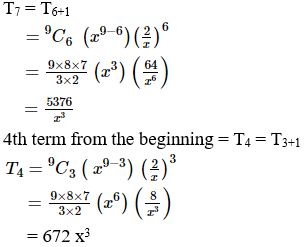7. Find the 4th term from the end in the expansion of (4x/5 – 5/2x) 9.

Solution:

Given:

(4x/5 – 5/2x) 9

Let Tr+1 be the 4th term from the end of the given expression.

Then, Tr+1 is (10 − 4 + 1)th term, i.e., 7th term, from the beginning.

T7 = T6+1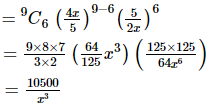∴ The 4th term from the end is 10500/x3.

8. Find the 7th term from the end in the expansion of (2x2 – 3/2x) 8.

Solution:

Given:

(2x2 – 3/2x) 8

Let Tr+1 be the 4th term from the end of the given expression.

Then, Tr+1 is (9 − 7 + 1)th term, i.e., 3rd term, from the beginning.

T3 = T2+1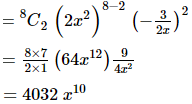∴ The 7th term from the end is 4032 x10.

9. Find the coefficient of:

(i)  x10 in the expansion of (2x2 – 1/x)20

(ii) x7 in the expansion of (x – 1/x2)40

(iii) x-15 in the expansion of (3x2 – a/3x3)10

(iv) x9 in the expansion of (x2 – 1/3x)9

(v) xm in the expansion of (x + 1/x)n

(vi) x in the expansion of (1 – 2x3 + 3x5) (1 + 1/x)8

(vii) a5b7 in the expansion of (a – 2b)12

(viii) x in the expansion of (1 – 3x + 7x2) (1 – x)16

Solution:

(i)  x10 in the expansion of (2x2 – 1/x)20

Given:

(2x2 – 1/x)20

If  x10 occurs in the (r + 1)th term in the given expression.

Then, we have:

Tr+1 = nCr xn-r ar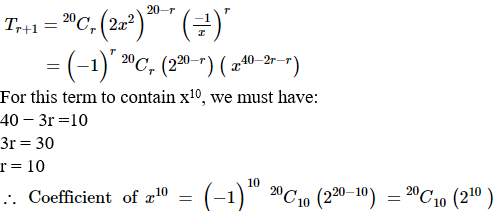(ii) x7 in the expansion of (x – 1/x2)40

Given:

(x – 1/x2)40

If xoccurs at the (r + 1) th term in the given expression.

Then, we have:

Tr+1 = nCr xn-r ar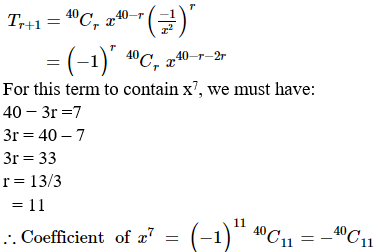40 − 3r =7

3r = 40 – 7

3r = 33

r = 33/3

= 11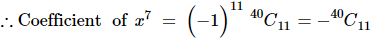(iii) x-15 in the expansion of (3x2 – a/3x3)10

Given:

(3x2 – a/3x3)10

If x−15 occurs at the (r + 1)th term in the given expression.

Then, we have:

Tr+1 = nCr xn-r ar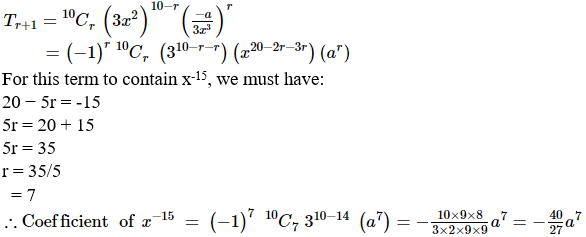(iv) x9 in the expansion of (x2 – 1/3x)9

Given:

(x2 – 1/3x)9

If x9 occurs at the (r + 1)th term in the above expression.

Then, we have:

Tr+1 = nCr xn-r ar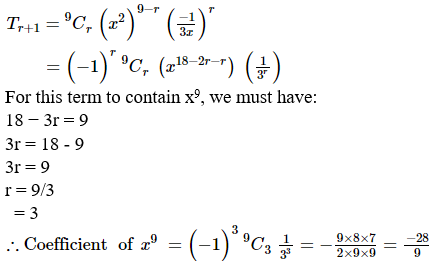For this term to contain x9, we must have:

18 − 3r = 9

3r = 18 – 9

3r = 9

r = 9/3

= 3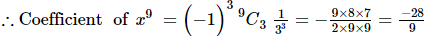(v) xm in the expansion of (x + 1/x)n

Given:

(x + 1/x)n

If xm occurs at the (r + 1)th term in the given expression.

Then, we have:

Tr+1 = nCr xn-r ar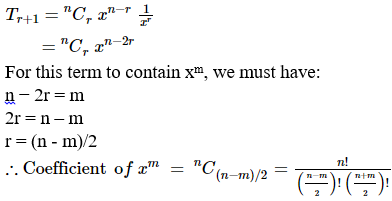(vi) x in the expansion of (1 – 2x3 + 3x5) (1 + 1/x)8

Given:

(1 – 2x3 + 3x5) (1 + 1/x)8

If x occurs at the (r + 1)th term in the given expression.

Then, we have:

(1 – 2x3 + 3x5) (1 + 1/x)8 = (1 – 2x3 + 3x5) (8C0 + 8C1 (1/x) + 8C2 (1/x)2 + 8C3 (1/x)3 + 8C4 (1/x)4 + 8C5 (1/x)5 + 8C6 (1/x)6 + 8C7 (1/x)7 + 8C8 (1/x)8)

So, ‘x’ occurs in the above expression at -2x3.8C2 (1/x2) + 3x5.8C4 (1/x4)

∴ Coefficient of x = -2 (8!/(2!6!)) + 3 (8!/(4! 4!))

= -56 + 210

= 154

(vii) a5b7 in the expansion of (a – 2b)12

Given:

(a – 2b)12

If a5b7 occurs at the (r + 1)th term in the given expression.

Then, we have:

Tr+1 = nCr xn-r ar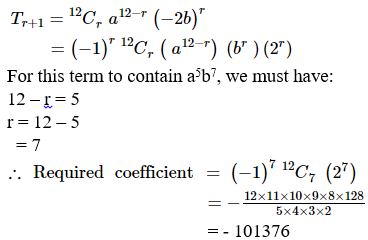(viii) x in the expansion of (1 – 3x + 7x2) (1 – x)16

Given:

(1 – 3x + 7x2) (1 – x)16

If x occurs at the (r + 1)th term in the given expression.

Then, we have:

(1 – 3x + 7x2) (1 – x)16 = (1 – 3x + 7x2) (16C0 + 16C1 (-x) + 16C2 (-x)2 + 16C3 (-x)3 + 16C4 (-x)4 + 16C5 (-x)5 + 16C6 (-x)6 + 16C7 (-x)7 + 16C8 (-x)8 + 16C9 (-x)9 + 16C10 (-x)10 + 16C11 (-x)11 + 16C12 (-x)12 + 16C13 (-x)13 + 16C14 (-x)14 + 16C15 (-x)15 + 16C16 (-x)16)

So, ‘x’ occurs in the above expression at 16C1 (-x) – 3x16C0

∴ Coefficient of x = -(16!/(1! 15!)) – 3(16!/(0! 16!))

= -16 – 3

= -19

10. Which term in the expansion of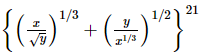contains x and y to one and the same power.

Solution:

Let us consider Tr+1 th term in the given expansion contains x and y to one and the same power.

Then we have,

Tr+1 = nCr xn-r ar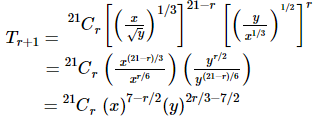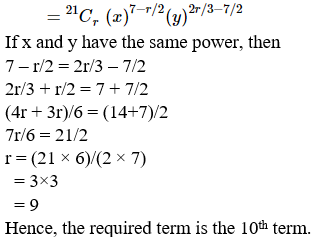11. Does the expansion of (2x2 – 1/x) contain any term involving x9?

Solution:

Given:

(2x2 – 1/x)

If x9 occurs at the (r + 1)th term in the given expression.

Then, we have:

Tr+1 = nCr xn-r ar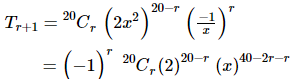For this term to contain x9, we must have

40 – 3r = 9

3r = 40 – 9

3r = 31

r = 31/3

It is not possible, since r is not an integer.

Hence, there is no term with x9 in the given expansion.

12. Show that the expansion of (x2 + 1/x)12 does not contain any term involving x-1.

Solution:

Given:

(x2 + 1/x)12

If x-1 occurs at the (r + 1)th term in the given expression.

Then, we have:

Tr+1 = nCr xn-r ar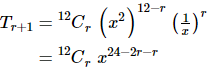For this term to contain x-1, we must have

24 – 3r = -1

3r = 24 + 1

3r = 25

r = 25/3

It is not possible, since r is not an integer.

Hence, there is no term with x-1 in the given expansion.

13. Find the middle term in the expansion of:

(i) (2/3x – 3/2x)20

(ii) (a/x + bx)12

(iii) (x2 – 2/x)10

(iv) (x/a – a/x)10

Solution:

(i) (2/3x – 3/2x)20

We have,

(2/3x – 3/2x)20 where, n = 20 (even number)

So the middle term is (n/2 + 1) = (20/2 + 1) = (10 + 1) = 11. ie., 11th term

Now,

T11 = T10+1

= 20C10 (2/3x)20-10 (3/2x)10

= 20C10 210/310 × 310/210 x10-10

= 20C10

Hence, the middle term is 20C10.

(ii) (a/x + bx)12

We have,

(a/x + bx)12 where, n = 12 (even number)

So the middle term is (n/2 + 1) = (12/2 + 1) = (6 + 1) = 7. ie., 7th term

Now,

T7 = T6+1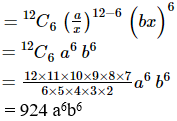= 924 a6b6

Hence, the middle term is 924 a6b6.

(iii) (x2 – 2/x)10

We have,

(x2 – 2/x)10 where, n = 10 (even number)

So the middle term is (n/2 + 1) = (10/2 + 1) = (5 + 1) = 6. ie., 6th term

Now,

T6 = T5+1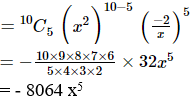Hence, the middle term is -8064x5.

(iv) (x/a – a/x)10

We have,

(x/a – a/x) 10 where, n = 10 (even number)

So the middle term is (n/2 + 1) = (10/2 + 1) = (5 + 1) = 6. ie., 6th term

Now,

T6 = T5+1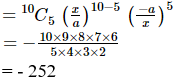Hence, the middle term is -252.

14. Find the middle terms in the expansion of:

(i) (3x – x3/6)9

(ii) (2x2 – 1/x)7

(iii) (3x – 2/x2)15

(iv) (x4 – 1/x3)11

Solution:

(i) (3x – x3/6)9

We have,

(3x – x3/6)9 where, n = 9 (odd number)

So the middle terms are ((n+1)/2) = ((9+1)/2) = 10/2 = 5 and

((n+1)/2 + 1) = ((9+1)/2 + 1) = (10/2 + 1) = (5 + 1) = 6

The terms are 5th and 6th.

Now,

T5 = T4+1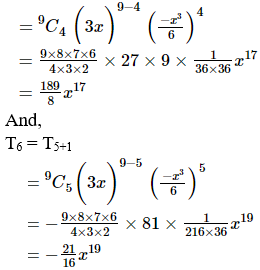Hence, the middle term are 189/8 x17 and -21/16 x19.

(ii) (2x2 – 1/x)7

We have,

(2x2 – 1/x)7 where, n = 7 (odd number)

So the middle terms are ((n+1)/2) = ((7+1)/2) = 8/2 = 4 and

((n+1)/2 + 1) = ((7+1)/2 + 1) = (8/2 + 1) = (4 + 1) = 5

The terms are 4th and 5th.

Now,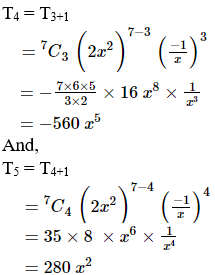Hence, the middle term are -560x5 and 280x2.

(iii) (3x – 2/x2)15

We have,

(3x – 2/x2)15 where, n = 15 (odd number)

So the middle terms are ((n+1)/2) = ((15+1)/2) = 16/2 = 8 and

((n+1)/2 + 1) = ((15+1)/2 + 1) = (16/2 + 1) = (8 + 1) = 9

The terms are 8th and 9th.

Now,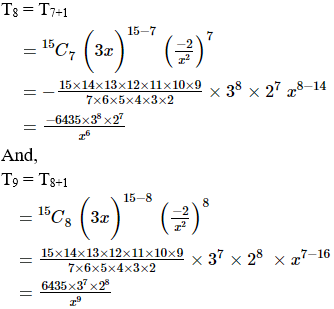Hence, the middle term are (-6435×38×27)/x6 and (6435×37×28)/x9.

(iv) (x4 – 1/x3)11

We have,

(x4 – 1/x3)11

where, n = 11 (odd number)

So the middle terms are ((n+1)/2) = ((11+1)/2) = 12/2 = 6 and

((n+1)/2 + 1) = ((11+1)/2 + 1) = (12/2 + 1) = (6 + 1) = 7

The terms are 6th and 7th.

Now,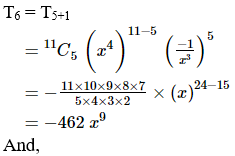T7 = T6+1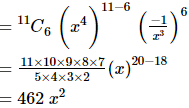Hence, the middle term are -462x9 and 462x2.

15. Find the middle terms in the expansion of:

(i) (x – 1/x)10

(ii) (1 – 2x + x2)n

(iii) (1 + 3x + 3x2 + x3)2n

(iv) (2x – x2/4)9

(v) (x – 1/x)2n+1

(vi) (x/3 + 9y)10

(vii) (3 – x3/6)7

(viii) (2ax – b/x2)12

(ix) (p/x + x/p)9

(x) (x/a – a/x)10

Solution:

(i) (x – 1/x)10

We have,

(x – 1/x)10 where, n = 10 (even number)

So the middle term is (n/2 + 1) = (10/2 + 1) = (5 + 1) = 6. ie., 6th term

Now,

T6 = T5+1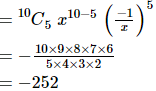Hence, the middle term is -252.

(ii) (1 – 2x + x2)n

We have,

(1 – 2x + x2)n = (1 – x)2n where, n is an even number.

So the middle term is (2n/2 + 1) = (n + 1)th term.

Now,

Tn = Tn+1

= 2nCn (-1)n (x)n

= (2n)!/(n!)2 (-1)n xn

Hence, the middle term is (2n)!/(n!)2 (-1)n xn.

(iii) (1 + 3x + 3x2 + x3)2n

We have,

(1 + 3x + 3x2 + x3)2n = (1 + x)6n where, n is an even number.

So the middle term is (n/2 + 1) = (6n/2 + 1) = (3n + 1)th term.

Now,

T2n = T3n+1

= 6nC3n x3n

= (6n)!/(3n!)2 x3n

Hence, the middle term is (6n)!/(3n!)2 x3n.

(iv) (2x – x2/4)9

We have,

(2x – x2/4)9 where, n = 9 (odd number)

So the middle terms are ((n+1)/2) = ((9+1)/2) = 10/2 = 5 and

((n+1)/2 + 1) = ((9+1)/2 + 1) = (10/2 + 1) = (5 + 1) = 6

The terms are 5th and 6th.

Now,

T5 = T4+1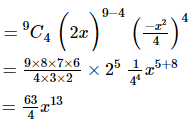And,

T6 = T5+1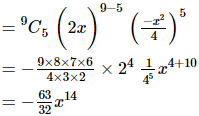Hence, the middle term is 63/4 x13 and -63/32 x14.

(v) (x – 1/x)2n+1

We have,

(x – 1/x)2n+1 where, n = (2n + 1) is an (odd number)

So the middle terms are ((n+1)/2) = ((2n+1+1)/2) = (2n+2)/2 = (n + 1) and

((n+1)/2 + 1) = ((2n+1+1)/2 + 1) = ((2n+2)/2 + 1) = (n + 1 + 1) = (n + 2)

The terms are (n + 1)th and (n + 2)th.

Now,

Tn = Tn+1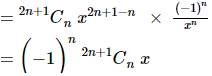And,

Tn+2 = Tn+1+1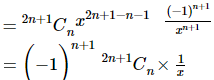Hence, the middle term is (-1)n.2n+1Cn x and (-1)n+1.2n+1Cn (1/x).

(vi) (x/3 + 9y)10

We have,

(x/3 + 9y)10 where, n = 10 is an even number.

So the middle term is (n/2 + 1) = (10/2 + 1) = (5 + 1) = 6. i.e., 6th term.

Now,

T6 = T5+1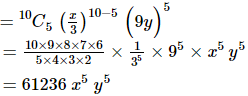Hence, the middle term is 61236x5y5.

(vii) (3 – x3/6)7

We have,

(3 – x3/6)7 where, n = 7 (odd number).

So the middle terms are ((n+1)/2) = ((7+1)/2) = 8/2 = 4 and

((n+1)/2 + 1) = ((7+1)/2 + 1) = (8/2 + 1) = (4 + 1) = 5

The terms are 4th and 5th.

Now,

T4 = T3+1

= 7C3 (3)7-3 (-x3/6)3

= -105/8 x9

And,

T5 = T4+1

= 9C4 (3)9-4 (-x3/6)4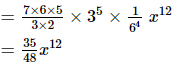Hence, the middle terms are -105/8 x9 and 35/48 x12.

(viii) (2ax – b/x2)12

We have,

(2ax – b/x2)12 where, n = 12 is an even number.

So the middle term is (n/2 + 1) = (12/2 + 1) = (6 + 1) = 7. i.e., 7th term.

Now,

T7 = T6+1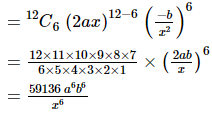Hence, the middle term is (59136a6b6)/x6.

(ix) (p/x + x/p)9

We have,

(p/x + x/p)9 where, n = 9 (odd number).

So the middle terms are ((n+1)/2) = ((9+1)/2) = 10/2 = 5 and

((n+1)/2 + 1) = ((9+1)/2 + 1) = (10/2 + 1) = (5 + 1) = 6

The terms are 5th and 6th.

Now,

T5 = T4+1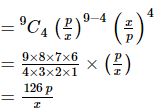And,

T6 = T5+1

= 9C5 (p/x)9-5 (x/p)5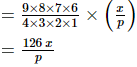Hence, the middle terms are 126p/x and 126x/p.

(x) (x/a – a/x)10

We have,

(x/a – a/x) 10 where, n = 10 (even number)

So the middle term is (n/2 + 1) = (10/2 + 1) = (5 + 1) = 6. ie., 6th term

Now,

T6 = T5+1Hence, the middle term is -252.

16. Find the term independent of x in the expansion of the following expressions:

(i) (3/2 x2 – 1/3x)9

(ii) (2x + 1/3x2)9

(iii) (2x2 – 3/x3)25

(iv) (3x – 2/x2)15

(v) ((√x/3) + √3/2x2)10

(vi) (x – 1/x2)3n

(vii) (1/2 x1/3 + x-1/5)8

(viii) (1 + x + 2x3) (3/2x2 – 3/3x)9

(ix) (∛x + 1/2∛x)18, x > 0

(x) (3/2x2 – 1/3x)6

Solution:

(i) (3/2 x2 – 1/3x)9

Given:

(3/2 x2 – 1/3x)9

If (r + 1)th term in the given expression is independent of x.

Then, we have:

Tr+1 = nCr xn-r ar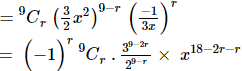For this term to be independent of x, we must have

18 – 3r = 0

3r = 18

r = 18/3

= 6

So, the required term is 7th term.

We have,

T7 = T6+1

= 9C6 × (39-12)/(29-6)

= (9×8×7)/(3×2) × 3-3 × 2-3

= 7/18

Hence, the term independent of x is 7/18.

(ii) (2x + 1/3x2)9

Given:

(2x + 1/3x2)9

If (r + 1)th term in the given expression is independent of x.

Then, we have:

Tr+1 = nCr xn-r ar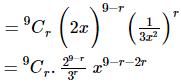For this term to be independent of x, we must have

9 – 3r = 0

3r = 9

r = 9/3

= 3

So, the required term is 4th term.

We have,

T4 = T3+1

= 9C3 × (26)/(33)

= 9C3 × 64/27

Hence, the term independent of x is 9C3 × 64/27.

(iii) (2x2 – 3/x3)25

Given:

(2x2 – 3/x3)25

If (r + 1)th term in the given expression is independent of x.

Then, we have:

Tr+1 = nCr xn-r ar

= 25Cr (2x2)25-r (-3/x3)r

= (-1)r 25Cr × 225-r × 3r x50-2r-3r

For this term to be independent of x, we must have

50 – 5r = 0

5r = 50

r = 50/5

= 10

So, the required term is 11th term.

We have,

T11 = T10+1

= (-1)10 25C10 × 225-10 × 310

= 25C10 (215 × 310)

Hence, the term independent of x is 25C10 (215 × 310).

(iv) (3x – 2/x2)15

Given:

(3x – 2/x2)15

If (r + 1)th term in the given expression is independent of x.

Then, we have:

Tr+1 = nCr xn-r ar

= 15Cr (3x)15-r (-2/x2)r

= (-1)r 15Cr × 315-r × 2r x15-r-2r

For this term to be independent of x, we must have

15 – 3r = 0

3r = 15

r = 15/3

= 5

So, the required term is 6th term.

We have,

T6 = T5+1

= (-1)5 15C5 × 315-5 × 25

= -3003 × 310 × 25

Hence, the term independent of x is -3003 × 310 × 25.

(v) ((√x/3) + √3/2x2)10

Given:

((√x/3) + √3/2x2)10

If (r + 1)th term in the given expression is independent of x.

Then, we have:

Tr+1 = nCr xn-r ar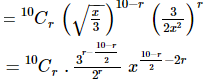For this term to be independent of x, we must have

(10-r)/2 – 2r = 0

10 – 5r = 0

5r = 10

r = 10/5

= 2

So, the required term is 3rd term.

We have,

T3 = T2+1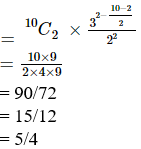Hence, the term independent of x is 5/4.

(vi) (x – 1/x2)3n

Given:

(x – 1/x2)3n

If (r + 1)th term in the given expression is independent of x.

Then, we have:

Tr+1 = nCr xn-r ar

= 3nCr x3n-r (-1/x2)r

= (-1)r 3nCr x3n-r-2r

For this term to be independent of x, we must have

3n – 3r = 0

r = n

So, the required term is (n+1)th term.

We have,

(-1)n 3nCn

Hence, the term independent of x is (-1)n 3nCn

(vii) (1/2 x1/3 + x-1/5)8

Given:

(1/2 x1/3 + x-1/5)8

If (r + 1)th term in the given expression is independent of x.

Then, we have:

Tr+1 = nCr xn-r ar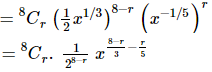For this term to be independent of x, we must have

(8-r)/3 – r/5 = 0

(40 – 5r – 3r)/15 = 0

40 – 5r – 3r = 0

40 – 8r = 0

8r = 40

r = 40/8

= 5

So, the required term is 6th term.

We have,

T6 = T5+1

= 8C5 × 1/(28-5)

= (8×7×6)/(3×2×8)

= 7

Hence, the term independent of x is 7.

(viii) (1 + x + 2x3) (3/2x2 – 3/3x)9

Given:

(1 + x + 2x3) (3/2x2 – 3/3x)9

If (r + 1)th term in the given expression is independent of x.

Then, we have:

(1 + x + 2x3) (3/2x2 – 3/3x)9 =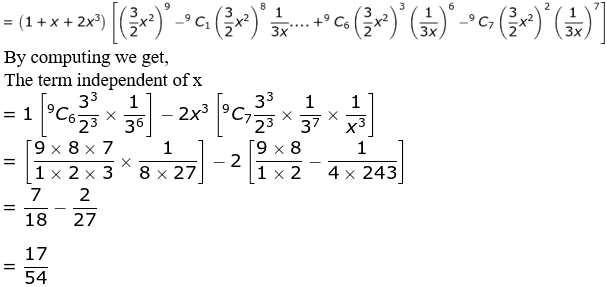= 7/18 – 2/27

= (189 – 36)/486

= 153/486 (divide by 9)

= 17/54

Hence, the term independent of x is 17/54.

(ix) (∛x + 1/2∛x)18, x > 0

Given:

(∛x + 1/2∛x)18, x > 0

If (r + 1)th term in the given expression is independent of x.

Then, we have:

Tr+1 = nCr xn-r ar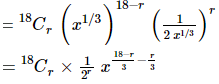For this term to be independent of r, we must have

(18-r)/3 – r/3 = 0

(18 – r – r)/3 = 0

18 – 2r = 0

2r = 18

r = 18/2

= 9

So, the required term is 10th term.

We have,

T10 = T9+1

= 18C9 × 1/29

Hence, the term independent of x is 18C9 × 1/29.

(x) (3/2x2 – 1/3x)6

Given:

(3/2x2 – 1/3x)6

If (r + 1)th term in the given expression is independent of x.

Then, we have:

Tr+1 = nCr xn-r ar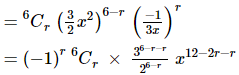For this term to be independent of r, we must have

12 – 3r = 0

3r = 12

r = 12/3

= 4

So, the required term is 5th term.

We have,

T5 = T4+1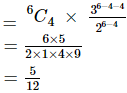Hence, the term independent of x is 5/12.

17. If the coefficients of (2r + 4)th and (r – 2)th terms in the expansion of (1 + x)18 are equal, find r.

Solution:

Given:

(1 + x)18

We know, the coefficient of the r term in the expansion of (1 + x)n is nCr-1

So, the coefficients of the (2r + 4) and (r – 2) terms in the given expansion are 18C2r+4-1 and 18Cr-2-1

For these coefficients to be equal, we must have

18C2r+4-1 = 18Cr-2-1

18C2r+3 = 18Cr-3

2r + 3 = r – 3 (or) 2r + 3 + r – 3 = 18 [Since, nCr = nCs => r = s (or) r + s = n]

2r – r = -3 – 3 (or) 3r = 18 – 3 + 3

r = -6 (or) 3r = 18

r = -6 (or) r = 18/3

r = -6 (or) r = 6

∴ r = 6 [since, r should be a positive integer.]

18. If the coefficients of (2r + 1)th term and (r + 2)th term in the expansion of (1 + x)43 are equal, find r.

Solution:

Given:

(1 + x)43

We know, the coefficient of the r term in the expansion of (1 + x)n is nCr-1

So, the coefficients of the (2r + 1) and (r + 2) terms in the given expansion are 43C2r+1-1 and 43Cr+2-1

For these coefficients to be equal, we must have

43C2r+1-1 = 43Cr+2-1

43C2r = 43Cr+1

2r = r + 1 (or) 2r + r + 1 = 43 [Since, nCr = nCs => r = s (or) r + s = n]

2r – r = 1 (or) 3r + 1 = 43

r = 1 (or) 3r = 43 – 1

r = 1 (or) 3r = 42

r = 1 (or) r = 42/3

r = 1 (or) r = 14

∴ r = 14 [since, value ‘1’ gives the same term]

19. Prove that the coefficient of (r + 1)th term in the expansion of (1 + x)n + 1 is equal to the sum of the coefficients of rth and (r + 1)th terms in the expansion of (1 + x)n.

Solution:

We know, the coefficients of (r + 1)th term in (1 + x)n+1 is n+1Cr

So, sum of the coefficients of the rth and (r + 1)th terms in (1 + x)n is

(1 + x)n = nCr-1 + nCr

= n+1Cr [since, nCr+1 + nCr = n+1Cr+1]

Hence proved.